# Coding

A crashcourse

Some parts of this course - especially the ones intended to familiarize you with the Bootstrap technique - may require a little bit of programming practice. To help with these parts, here is a very concise intro to just enough Python (with NumPy, SciPy, Pandas and Statsmodels) or R (with RStudio and Tidyverse) to get you through the exercises. There are plenty of tutorials and introductions to help you get acquainted with the whole programming language - this is intended as a cookbook style bare bones toolbox.

Download and install JetBrains’ PyCharm or DataSpell for a good development environment to write Python scripts and notebooks in. Your student status and campus email will get you an educational license for these tools.

R is the programming language itself, RStudio is a software developed specifically to make it easy to interact with R.

# Make sure all libraries are installed

Anaconda and PyCharm and DataSpell all have good interfaces to package managers. Search for, and make sure you have downloaded and installed, the following packages: numpy, scipy, pandas, matplotlib, seaborn, statsmodels.

Most likely, Google Colaboratory already has everything you need. If every you run into a package you try to load but does not seem to exist, write the following on its own in a code cell and execute it:

!pip install <package-name>

After the package has installed you can remove the cell again, you will not need to rerun the command.

In the Console part of the RStudio window (bottom left), run the following command:

install.packages(c("tidyverse", "foreach"))

The command install.packages will help you add missing libraries to R installations when you need them.

There are some circumstances that generate very many errors when trying to install tidyverse. This is often due to missing software on the computer, and it might be easier to switch to the Cloud solution for R computations.

In the Console part of the RStudio window (bottom left), run the following command:

install.packages(c("tidyverse", "foreach"))

The command install.packages will help you add missing libraries to R installations when you need them.

# Starting a new script / notebook / project

Create a new project / new notebook. In this notebook you will collect code cells with executable code, and markdown cells that you can fill with text explaining what you are doing and why.

In the first code cell of the project, put the following:

%matplotlib inline

import numpy as np
import scipy as sp
import pandas as pd
import statsmodels
import scipy.stats
from matplotlib import pyplot

Make sure you execute this code cell before you continue with the rest of your code.

Create a new R script (if you want to just write code) or a new RMarkdown or Quarto document (if you want to write text with code elements interspersed, like the lecture slides are written).

In RMarkdown or Quarto, you create code cells that contain R code that does computations by writing

{r}
# R code goes here


Whichever way you build your code (script or as a document with code-blocks), include the following lines of code at the very start of the script

library(tidyverse)
library(foreach)

Load from a CSV file on the internet (replace url-to-the-file.csv with the URL address for the file)

data = pd.read_csv("url-to-the-file.csv")

Load from a CSV file in your file system (replace path/to/file.csv with the path and file name for the file)

data = pd.read_csv("path/to/file.csv"

Enter data by hand

data = pd.DataFrame(
{
"variable1": [1,2,3,4,5,8],
"variable2": [1,4,9,16,25,64]
}
)

### Access data

Access an entire column

data.variable1
# or
data["variable1"]

Access a row

data.loc  # uses row name
data.iloc # uses row number (starting with 0)

Access a single value

data.variable1
data["variable1"]
data.loc["variable1"]
data.loc[2, "variable1"]
data.iloc[2, 0]

Load from a CSV file on the internet (replace url-to-the-file.csv with the URL address for the file)

data = read_csv("url-to-the-file.csv")

Load from a CSV file in your file system (replace path/to/file.csv with the path and file name for the file)

data = read_csv("path/to/file.csv"

Enter data by hand

data = tibble(variable1=c(1,2,3,4,5,8),
variable2=c(1,4,9,16,25,64))

### Access data

Access an entire column

data$variable1 # or data["variable1"] # or data # note: starting with 1 Access a row data[2,] # note: row numbers start with 1 Access a single value data$variable1
# or
data[2,"variable1"]
# or
data[2,1]

# Compute PDF, CDF, random values, etc

Use the scipy.stats objects (see https://docs.scipy.org/doc/scipy/reference/stats.html:

Continuous Distributions:

• scipy.stats.chi2(df) - the chi-squared distribution
• scipy.stats.expon(scale=1/lambda) - the exponential distribution
• scipy.stats.f(dfn, dfd) - the F distribution (with degrees of freedom for numerator and denominator)
• scipy.stats.gamma(alpha, scale=1/beta) - the Gamma distribution (with shape, scale parameters, not shape, rate)
• scipy.stats.lognorm(s=sigma, scale=np.exp(mu)) - the Log-Normal distribution, so that log(x) is $$\mathcal{N}(\mu,\sigma^2)$$
• scipy.stats.nct(df, ncp) - the non-central t-distribution
• scipy.stats.norm(loc=mu, scale=sigma) - the normal distribution with mean mu and standard deviation (not variance) sigma
• scipy.stats.t(df) - Student’s T distribution
• scipy.stats.uniform(loc=a, scale=b-a) - the uniform distribution with min given as loc and width as scale

Multivariate Continuous Distributions:

• scipy.stats.multivariate_normal(mean=mu, cov=covariance) - multivariate normal distribution with stated mean vector and covariance matrix
• scipy.stats.multivariate_t(df) - multivariate T-distribution

Empirical Distribution:

hist = np.histogram(data.variable, density=False)
dist = scipy.stats.rv_histogram(hist, density=False)

All these have functions

• rvs(n) - generate $$n$$ random numbers
• cdf(x) - compute $$CDF(x)$$ (lower tail prob.)
• ppf(x) - compute $$CDF^{-1}(x)$$ (lower tail quantile)
• sf(x) - compute $$1-CDF(x)$$ (upper tail prob.)
• isf(x) - compute $$CDF^{-1}(1-x)$$ (upper tail quantile)
• pdf(x) - compute $$PDF(x)$$
• logpdf(x) - compute $$\log PDF(x)$$
• logcdf(x) - compute $$\log CDF(x)$$
• logsf(x) - compute $$\log (1-CDF(x))$$

(as well as a number of functions for computing population statistics, entropy, moments, and fitting the distribution to observed data)

Discrete Distributions:

• scipy.stats.bernoulli(p) - the Bernoulli distribution
• scipy.stats.binom(n,p) - the Binomial distribution
• scipy.stats.geom(p) - the geometric distribution, ie # failures before first success
• scipy.stats.hypergeom(M, n, N) - the hypergeometric distribution; In an urn with $$M$$ balls, $$n$$ white and $$M-n$$ black, count how many white balls are drawn when drawing $$N$$ without replacement
• scipy.stats.nbinom(n,p) - the negative binomial distribution counting number of failures before $$n$$ successes happen
• scipy.stats.poisson(mu) - the Poisson distribution
• scipy.stats.randint(low, high) - the discrete uniform distribution

Multivariate Discrete Distributions:

• scipy.stats.multinomial(n, [p1,p2,...,pm]) - multinomial distribution with probabilities $$p_1,\dots,p_m$$ for the $$m$$ different categories
• scipy.stats.multivariate_hypergeom([m1,m2,...,mk], n) - multivariate hypergeometric distribution where there are $$n$$ samples taken from a population with $$m_i$$ objects of the i:th type.

All these have functions

• rvs(n) - generate $$n$$ random numbers
• cdf(x) - compute $$CDF(x)$$ (lower tail prob.)
• ppf(x) - compute $$CDF^{-1}(x)$$ (lower tail quantile)
• sf(x) - compute $$1-CDF(x)$$ (upper tail prob.)
• isf(x) - compute $$CDF^{-1}(1-x)$$ (upper tail quantile)
• pmf(x) - compute $$\PP(x)$$
• logpdf(x) - compute $$\log PDF(x)$$
• logcdf(x) - compute $$\log CDF(x)$$
• logsf(x) - compute $$\log (1-CDF(x))$$

(as well as a number of functions for computing population statistics, entropy, moments, and fitting the distribution to observed data)

Each distribution defines functions:

• r? - generate random numbers
• d? - $$PDF$$
• p? - $$CDF$$
• q? - $$CDF^{-1}$$

Commonly used distributions include (replace ? with r/d/p/q and with the input [number of random values; x; x; p])

• ?binom(?, n, p) - Binomial and Bernoulli
• ?chisq(?, df) - Chi-square
• ?exp(?, lambda) - Exponential
• ?f(?, df1, df2) - F
• ?gamma(?, shape, scale=?) or ?gamma(?, shape, rate=?) - Gamma
• ?geom(?, p) - Geometric, ie # failures before first success
• ?hyper(?, m, n, k) - Hypergeometric, drawing $$k$$ balls from $$m$$ white and $$n$$ black balls in an urn, and counting the number of white balls drawn
• ?lnorm(?, meanlog=mu, sdlog=sigma) - Log normal
• rmultinom(n, size, c(p1, p2, ..., pm)), dmultinom(c(x1, x2, ..., xm), prob=c(p1, p2, ..., pm)) - random values and probability density for the multinomial (multivariate) distribution where the probability of drawing the i:th type of object is pi
• ?nbinom(?, n, p) - negative binomial
• ?norm(?, mean=mu, sd=sigma) - normal; note that it takes standard deviations and not variances
• ?pois(?, lambda) - Poisson
• ?t(?, df) - Student’s T
• ?t(?, df, ncp) - Non-central Student’s T; no random values, only available if abs(ncp) <= 37.62
• ?unif(?, min=a, max=b) - Uniform

# Bootstrap Sample

We use the data.sample method from Pandas with a list comprehension to quickly compute a bootstrap sample:

bootstrap_samples = [data.sample(frac=1.0, replace=True).variable1.median() for i in range(999)]

Replace .median to get a different statistic (Pandas provides kurtosis, mad (mean absolute deviation), max, mean, median, min, mode, product, quantile, rank, sem (standard error of the mean), skew, sum, std, var, nunique; std, var, skew and kurtosis are unbiased estimator)

Replace 999 with the number of samples you want to generate.

Alternatively, for some other function f, you can use:

bootstrap_samples = [f(data.sample(frac=1.0, replace=True).variable1) for i in range(999)]

The result is a list (not a Pandas DataFrame), can be used with for instance np.quantile to compute bootstrap quantiles, np.mean and np.std for means and standard deviations, etc.

This code sample uses the foreach package for iteration; there are many other ways to do the same thing. It also extensively uses the tidyverse toolkit.

bootstrap_samples = foreach(i=1:999, .combine=rbind) %do% (
data %>% sample_frac(replace=TRUE) %>% summarise(stat=median(variable1))
)

Replace median to get a different statistic.

Replace 999 with the number of samples you want to generate.

# Bootstrap Using Utility Functions

These may require you to write small functions to fully specify the computation you need to perform.

SciPy provides the scipy.stats.bootstrap function:

bootstrap_sample = scipy.stats.bootstrap(data.variable1, np.median)

(the bootstrap function expects a sequence of arrays to sample from)

Printing the object reports the confidence interval and standard error. You can access the sample itself as bootstrap_sample.bootstrap_distribution.

The system will happily compute 3 different kinds of bootstrap intervals for you:

scipy.stats.bootstrap(data.variable1, np.median, method="percentile")
scipy.stats.bootstrap(data.variable1, np.median, method="basic")
scipy.stats.bootstrap(data.variable1, np.median, method="BCa")

If you want to change confidence level, method, or other additional tasks, you can also hand in a previously computed bootstrap_sample into the bootstrap function:

scipy.stats.bootstrap(data.variable1, np.median, method="basic", bootstrap_result=bootstrap_sample)

The library boot (install using install.packages if it is missing) provides a function for orchestrating bootstrap computations. You will need to write a function to perform the computation on a bootstrap sample, but the included code can be extensively re-used.

library(boot)

stat = function(data, indices) {
datasample = data[indices,] # pick out the elements chosen by the bootstrap
return(median(datasample$variable)) } bootstrap_output = boot(data, stat) You can then query this bootstrap_output object for additional information: bootstrap$t0 is the (set of) statistics applied to the original data and bootstrapt is a matrix where each row is a bootstrap replicate of all the statistics. print(bootstrap_output) and plot(bootstrap_output) both produce helpful summary outputs. To access confidence intervals, use the boot.ci function, which takes the output as well as conf=0.95 for the confidence level (default is 0.95) and type="all" for the confidence interval type (with options "norm", "basic", "stud", "perc", "bca", and "all") # Pivotting To move between long format data sets and wide format data sets, there are functions that help with the conversion. In a long format data set, values are in one column while their corresponding labels live in a different column – whereas in a wide format data sets, each label becomes a column of its own carrying the values directly. pandas provides a function DataFrame.pivot and DataFrame.melt that can be used for this. Let’s take a data set: Code import pandas as pd import numpy as np import scipy as sp df = pd.DataFrame({ "wheat": [5.2, 4.5, 6.0, 6.1, 6.7, 5.8], "barley": [6.5, 8.0, 6.1, 7.5, 5.9, 5.6], "maize": [5.8, 4.7, 6.4, 4.9, 6.0, 5.2], "oats": [8.3, 6.1, 7.8, 7.0, 5.5, 7.2] }) df  wheat barley maize oats 0 5.2 6.5 5.8 8.3 1 4.5 8.0 4.7 6.1 2 6.0 6.1 6.4 7.8 3 6.1 7.5 4.9 7.0 4 6.7 5.9 6.0 5.5 5 5.8 5.6 5.2 7.2 To get to a long format, use melt: Code df_long = df.melt() df_long  variable value 0 wheat 5.2 1 wheat 4.5 2 wheat 6.0 3 wheat 6.1 4 wheat 6.7 5 wheat 5.8 6 barley 6.5 7 barley 8.0 8 barley 6.1 9 barley 7.5 10 barley 5.9 11 barley 5.6 12 maize 5.8 13 maize 4.7 14 maize 6.4 15 maize 4.9 16 maize 6.0 17 maize 5.2 18 oats 8.3 19 oats 6.1 20 oats 7.8 21 oats 7.0 22 oats 5.5 23 oats 7.2 To get back to a wide format, use pivot: Code df_wide = df_long.pivot(columns="variable") df_wide  value variable barley maize oats wheat 0 NaN NaN NaN 5.2 1 NaN NaN NaN 4.5 2 NaN NaN NaN 6.0 3 NaN NaN NaN 6.1 4 NaN NaN NaN 6.7 5 NaN NaN NaN 5.8 6 6.5 NaN NaN NaN 7 8.0 NaN NaN NaN 8 6.1 NaN NaN NaN 9 7.5 NaN NaN NaN 10 5.9 NaN NaN NaN 11 5.6 NaN NaN NaN 12 NaN 5.8 NaN NaN 13 NaN 4.7 NaN NaN 14 NaN 6.4 NaN NaN 15 NaN 4.9 NaN NaN 16 NaN 6.0 NaN NaN 17 NaN 5.2 NaN NaN 18 NaN NaN 8.3 NaN 19 NaN NaN 6.1 NaN 20 NaN NaN 7.8 NaN 21 NaN NaN 7.0 NaN 22 NaN NaN 5.5 NaN 23 NaN NaN 7.2 NaN For a more compact version, first add a row-number variable within each group: Code df_long["id"] = df_long.groupby("variable").cumcount() df_wide = df_long.pivot(columns="variable", index="id") df_wide  value variable barley maize oats wheat id 0 6.5 5.8 8.3 5.2 1 8.0 4.7 6.1 4.5 2 6.1 6.4 7.8 6.0 3 7.5 4.9 7.0 6.1 4 5.9 6.0 5.5 6.7 5 5.6 5.2 7.2 5.8 In tidyverse, we get the functions pivot_longer and pivot_wider, that can be used as follows: Code library(tidyverse) library(knitr) # From Exercise 11.8 df = tibble( wheat = c(5.2, 4.5, 6.0, 6.1, 6.7, 5.8), barley = c(6.5, 8.0, 6.1, 7.5, 5.9, 5.6), maize = c(5.8, 4.7, 6.4, 4.9, 6.0, 5.2), oats = c(8.3, 6.1, 7.8, 7.0, 5.5, 7.2) ) df %>% kable() wheat barley maize oats 5.2 6.5 5.8 8.3 4.5 8.0 4.7 6.1 6.0 6.1 6.4 7.8 6.1 7.5 4.9 7.0 6.7 5.9 6.0 5.5 5.8 5.6 5.2 7.2 pivot_longer moves us from wide to long. Tell pivot_longer which columns need to be recoded as a name column and as a value column. There are a number of helper functions in tidyverse to make these specifications much easier - such as everything() or starts_with("prefix") or ends_with("suffix") or contains("content"). You can also just list the columns that you want to rewrite. Code df_long = df %>% pivot_longer(everything()) df_long %>% kable() name value wheat 5.2 barley 6.5 maize 5.8 oats 8.3 wheat 4.5 barley 8.0 maize 4.7 oats 6.1 wheat 6.0 barley 6.1 maize 6.4 oats 7.8 wheat 6.1 barley 7.5 maize 4.9 oats 7.0 wheat 6.7 barley 5.9 maize 6.0 oats 5.5 wheat 5.8 barley 5.6 maize 5.2 oats 7.2 pivot_wider moves us from long to wide. You need to tell the function where the names live with names_from and where the values live with values_from, and it will construct new column names from these. If you want control over how the column names get constructed, you may want to use the arguments names_prefix, names_sep or names_glue. If you have any duplicate values, pivot_wider will struggle pivotting. The solution is to make sure you have an ID column, or by calling the unnest function to unwrap the lists pivot_wider creates. Code df_wide = df_long %>% pivot_wider(names_from=name, values_from=value) %>% unnest() df_wide %>% kable wheat barley maize oats 5.2 6.5 5.8 8.3 4.5 8.0 4.7 6.1 6.0 6.1 6.4 7.8 6.1 7.5 4.9 7.0 6.7 5.9 6.0 5.5 5.8 5.6 5.2 7.2 # Group-wise summaries and computations One very useful way to use long-form data frames is to do the same computations for all groups simultaneously, and extracting either a transformed or a summarized data frame. Both pandas and tidyverse have extensive support for these operations through a group by operation. The .groupby operation on a pandas data frame sets up a grouped data frame that can be used for aggregating and transforming data. First off, the .describe() method computes a collection of useful summary statistics. Code df_grouped = df_long.groupby("variable") df_grouped.value.describe()  count mean std min 25% 50% 75% max variable barley 6.0 6.600000 0.950789 5.6 5.950 6.3 7.250 8.0 maize 6.0 5.500000 0.669328 4.7 4.975 5.5 5.950 6.4 oats 6.0 6.983333 1.041953 5.5 6.325 7.1 7.650 8.3 wheat 6.0 5.716667 0.767898 4.5 5.350 5.9 6.075 6.7 There is a large collection of already defined and useful individual summary functions in pandas. Method Example .count() (also .size() - count excludes NA, size includes them in the count) df_grouped.value.count() variable barley 6 maize 6 oats 6 wheat 6 Name: value, dtype: int64 .first() (also .last()) df_grouped.first()  value id variable barley 6.5 0 maize 5.8 0 oats 8.3 0 wheat 5.2 0 .nth() (also .head() and .tail()) print(df_grouped.nth(2)) print(df_grouped.head(2))  value id variable barley 6.1 2 maize 6.4 2 oats 7.8 2 wheat 6.0 2  variable value id 0 wheat 5.2 0 1 wheat 4.5 1 6 barley 6.5 0 7 barley 8.0 1 12 maize 5.8 0 13 maize 4.7 1 18 oats 8.3 0 19 oats 6.1 1 .max() (also .min()) df_grouped.value.max() variable barley 8.0 maize 6.4 oats 8.3 wheat 6.7 Name: value, dtype: float64 .idxmax() (also .idxmin()) df_grouped.value.idxmax() variable barley 7 maize 14 oats 18 wheat 4 Name: value, dtype: int64 .mean() df_grouped.value.mean() variable barley 6.600000 maize 5.500000 oats 6.983333 wheat 5.716667 Name: value, dtype: float64 .std() (also .var()) df_grouped.value.std() variable barley 0.950789 maize 0.669328 oats 1.041953 wheat 0.767898 Name: value, dtype: float64 .sem() (standard error of the mean) df_grouped.value.sem() variable barley 0.388158 maize 0.273252 oats 0.425376 wheat 0.313493 Name: value, dtype: float64 .median() (also .quantile(q)) df_grouped.value.median() variable barley 6.3 maize 5.5 oats 7.1 wheat 5.9 Name: value, dtype: float64 .sum() (also .prod()) df_grouped.value.sum() variable barley 39.6 maize 33.0 oats 41.9 wheat 34.3 Name: value, dtype: float64 .nunique() df_grouped.value.nunique() variable barley 6 maize 6 oats 6 wheat 6 Name: value, dtype: int64 With the function .agg (short for .aggregate), you can both apply several different summary functions at once, and also apply functions not implemented in pandas. Code df_grouped.value.agg(["mean", "sem", np.ptp, "median", lambda x: np.quantile(x, 0.75)-np.quantile(x,0.25)]).rename( columns={"<lambda_0>": "iqr", "ptp": "range"} )  mean sem range median iqr variable barley 6.600000 0.388158 2.4 6.3 1.300 maize 5.500000 0.273252 1.7 5.5 0.975 oats 6.983333 0.425376 2.8 7.1 1.325 wheat 5.716667 0.313493 2.2 5.9 0.725 The tidyverse has a rich landscape of functions to transform datasets. Grouping and group-wise computations go through the group_by function: Code df_grouped = df_long %>% group_by(name) With a grouped data frame, we can use summarise to compute group-wise summaries with any function that operates on a sequence: df_grouped %>% summarise(mean=mean(value), sd=sd(value), median=median(value), IQR=IQR(value), mad=mad(value), max=max(value), argmax=which.max(value)) %>% kable() name mean sd median IQR mad max argmax barley 6.600000 0.9507891 6.3 1.300 0.81543 8.0 2 maize 5.500000 0.6693280 5.5 0.975 0.81543 6.4 3 oats 6.983333 1.0419533 7.1 1.325 1.26021 8.3 1 wheat 5.716667 0.7678976 5.9 0.725 0.66717 6.7 5 Here, IQR is the inter-quartile range and mad is the median absolute deviation. We can also filter our data within the groups Code df_grouped %>% filter(value > mean(value)) %>% arrange(.by_group=TRUE) %>% kable() name value barley 8.0 barley 7.5 maize 5.8 maize 6.4 maize 6.0 oats 8.3 oats 7.8 oats 7.0 oats 7.2 wheat 6.0 wheat 6.1 wheat 6.7 wheat 5.8 We can (for instance) resample the data independently in each group, for a multiple group bootstrap. Code df_grouped %>% slice_sample(prop=1.0, replace=TRUE) %>% summarise_all(median) # A tibble: 4 × 2 name value <chr> <dbl> 1 barley 7 2 maize 5.8 3 oats 7.75 4 wheat 5.5  # Permutation Testing For permutation testing we would permute group membership among the values, and then re-compute some quantity (like for instance group means and the group mean differences; or some energy function that captures some other behavior). With pandas, this can be a little bit tricky, because pandas works hard to maintain the row index of your data. So you’ll need to use reset_index to avoid the index alignment. Say that we want to find out if wheat or barley has a much higher median than the other. Code df_wb = df_long.query("variable in ['wheat','barley']") df_perm = pd.concat([df_wb.groupby("variable").value.median()] + [df_wb.apply( lambda x: x.sample(frac=1).values ).groupby("variable").value.median() for _ in range(999)], axis=1).T perm_diff = df_perm.barley-df_perm.wheat perm_diff.head(5) value 0.40 value -0.35 value 0.45 value 0.90 value -0.50 dtype: float64 Once you have a sample like this one, you can compute .rank() to figure out how extreme each value is in its respective column, which you will need to compute a p-value for the permutation test. By using .rank(method="max"), each rank counts how many elements (including the current one) have its value or less. Code (perm_diff.rank(method="max").head(1))/(perm_diff.size+1) value 0.835165 dtype: float64 Permutation testing in the tidyverse can easily be done by using sample from base R within a tidyverse mutate verb. Code df_long %>% filter(name %in% c("wheat","barley")) %>% mutate(name=sample(name)) %>% group_by(name) %>% summarise(median=median(value)) %>% reframe(median.diff=diff(median)) # A tibble: 1 × 1 median.diff <dbl> 1 -0.75 Once we have the code to do what we need to do once, we can use foreach to repeat it: Code library(foreach) median.diffs = rbind( df_long %>% filter(name %in% c("wheat","barley")) %>% group_by(name) %>% summarise(median=median(value)) %>% reframe(median.diff=diff(median)), # Our observed data foreach(i=1:999, .combine=rbind) %do% (df_long %>% filter(name %in% c("wheat","barley")) %>% mutate(name=sample(name)) %>% group_by(name) %>% summarise(median=median(value)) %>% reframe(median.diff=diff(median)) # Our permutations ) ) median.diffs %>% head(5) # A tibble: 5 × 1 median.diff <dbl> 1 -0.400 2 -0.5 3 0.400 4 -0.45 5 0.300 Now with these median differences from each permutation, we can use rank to compute a p-value. By using ties.method="min", each rank counts how many entries, itself excluded are that size or smaller. n() can be used to get the total number of observations. Code median.diffs %>% transmute(rank=rank(median.diff, ties.method="min"), p.value=rank/(n()+1)) %>% head(1) # A tibble: 1 × 2 rank p.value <int> <dbl> 1 155 0.155 # Linear Regression and ANOVA SciPy has support for linear regression through scipy.stats.linregress and for ANOVA through scipy.stats.f_oneway. import palmerpenguins penguins = palmerpenguins.load_penguins().dropna() penguins.head()  species island bill_length_mm ... body_mass_g sex year 0 Adelie Torgersen 39.1 ... 3750.0 male 2007 1 Adelie Torgersen 39.5 ... 3800.0 female 2007 2 Adelie Torgersen 40.3 ... 3250.0 female 2007 4 Adelie Torgersen 36.7 ... 3450.0 female 2007 5 Adelie Torgersen 39.3 ... 3650.0 male 2007 [5 rows x 8 columns] ### Linear Regression import scipy, scipy.stats model = scipy.stats.linregress(penguins.bill_length_mm, penguins.body_mass_g) print(model) LinregressResult(slope=86.79175964755551, intercept=388.84515876027217, rvalue=0.5894511101769491, pvalue=1.538613514485938e-32, stderr=6.53766608047017, intercept_stderr=289.817203328937) print(f"R^2: {model.rvalue**2}") R^2: 0.34745261128883775 from matplotlib import pyplot pyplot.figure() pyplot.plot(penguins.bill_length_mm, penguins.body_mass_g, 'o', label="data") pyplot.plot(penguins.bill_length_mm, model.intercept + model.slope*penguins.bill_length_mm, 'r', label="fitted line") pyplot.legend() pyplot.show()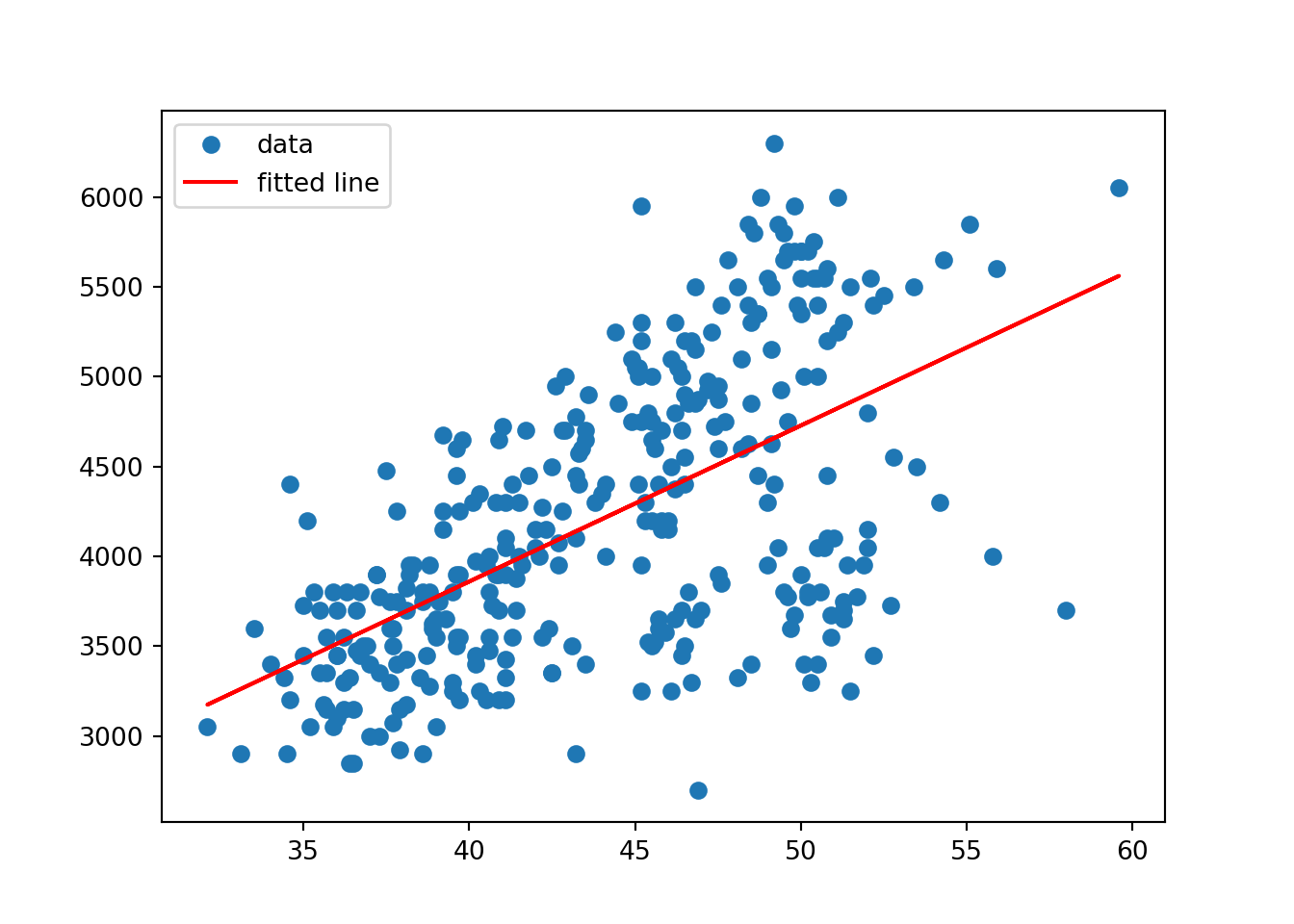### ANOVA Within a single species, are the body masses dependent on island? Only Adelie penguins were observed on all three islands. Let’s do an ANOVA. scipy.stats.f_oneway( penguins.query("species == 'Adelie'").query("island=='Biscoe'").body_mass_g, penguins.query("species == 'Adelie'").query("island=='Dream'").body_mass_g, penguins.query("species == 'Adelie'").query("island=='Torgersen'").body_mass_g) F_onewayResult(statistic=0.004838458443284365, pvalue=0.9951733909544992) How about the three different species, do they have different bill sizes? scipy.stats.f_oneway( penguins.query("species == 'Adelie'").bill_length_mm, penguins.query("species == 'Gentoo'").bill_length_mm, penguins.query("species == 'Chinstrap'").bill_length_mm ) F_onewayResult(statistic=397.29943741282796, pvalue=1.3809842053151752e-88) R has extensive support for linear regression through lm and for ANOVA through aov. The command anova is used to print out ANOVA tables for many kinds of models (including linear regression and ANOVA). ### Linear Regression library(tidyverse) library(ggfortify) library(palmerpenguins) model = lm(body_mass_g ~ bill_length_mm, data=penguins) model  Call: lm(formula = body_mass_g ~ bill_length_mm, data = penguins) Coefficients: (Intercept) bill_length_mm 362.31 87.42  summary(model)  Call: lm(formula = body_mass_g ~ bill_length_mm, data = penguins) Residuals: Min 1Q Median 3Q Max -1762.08 -446.98 32.59 462.31 1636.86 Coefficients: Estimate Std. Error t value Pr(>|t|) (Intercept) 362.307 283.345 1.279 0.202 bill_length_mm 87.415 6.402 13.654 <2e-16 *** --- Signif. codes: 0 '***' 0.001 '**' 0.01 '*' 0.05 '.' 0.1 ' ' 1 Residual standard error: 645.4 on 340 degrees of freedom (2 observations deleted due to missingness) Multiple R-squared: 0.3542, Adjusted R-squared: 0.3523 F-statistic: 186.4 on 1 and 340 DF, p-value: < 2.2e-16 anova(model) Analysis of Variance Table Response: body_mass_g Df Sum Sq Mean Sq F value Pr(>F) bill_length_mm 1 77669072 77669072 186.44 < 2.2e-16 *** Residuals 340 141638626 416584 --- Signif. codes: 0 '***' 0.001 '**' 0.01 '*' 0.05 '.' 0.1 ' ' 1 autoplot(model)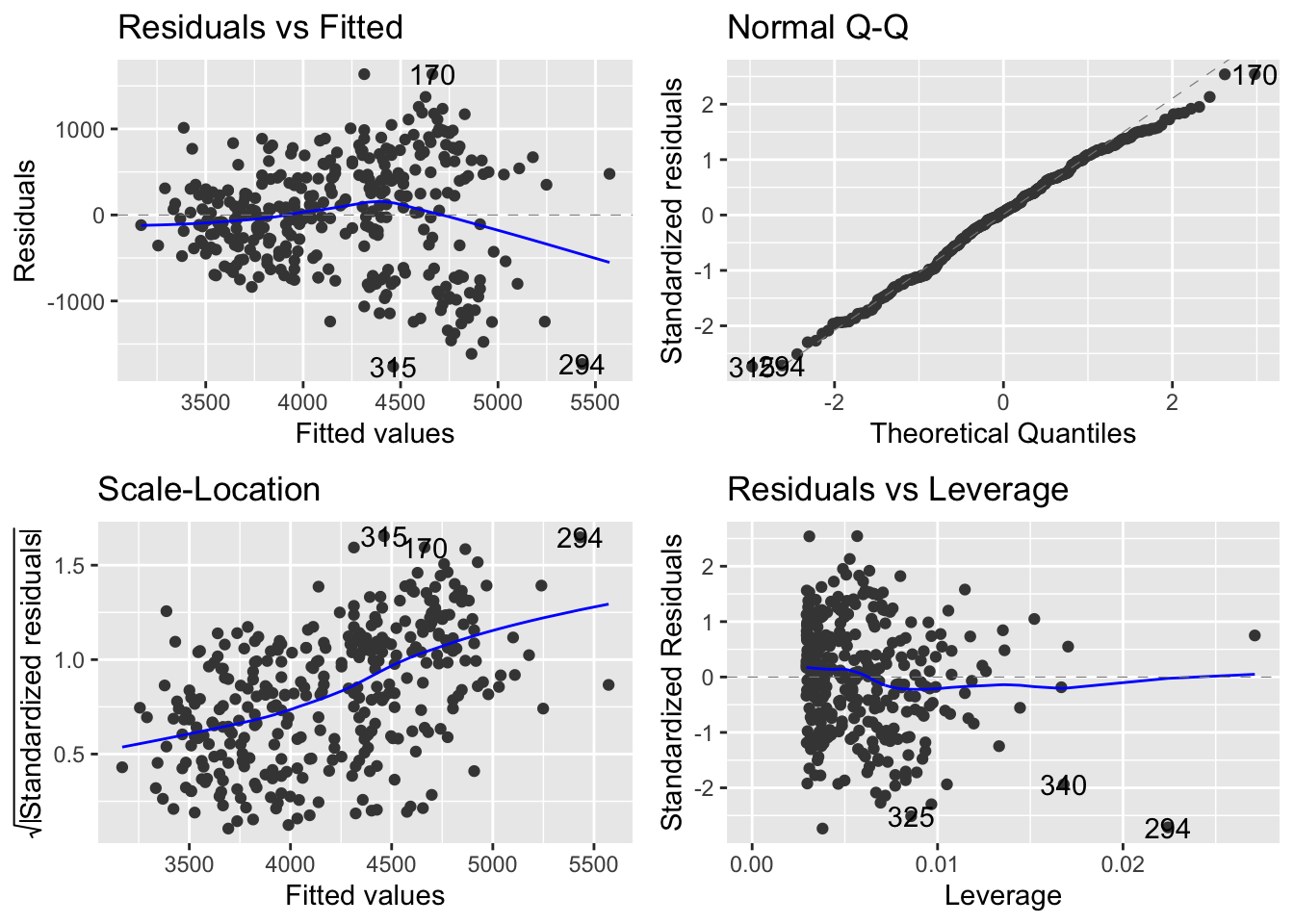ggplot(penguins, aes(bill_length_mm, body_mass_g)) + geom_point() + geom_smooth(method="lm") geom_smooth() using formula = 'y ~ x' Warning: Removed 2 rows containing non-finite values (stat_smooth()). Warning: Removed 2 rows containing missing values (geom_point()).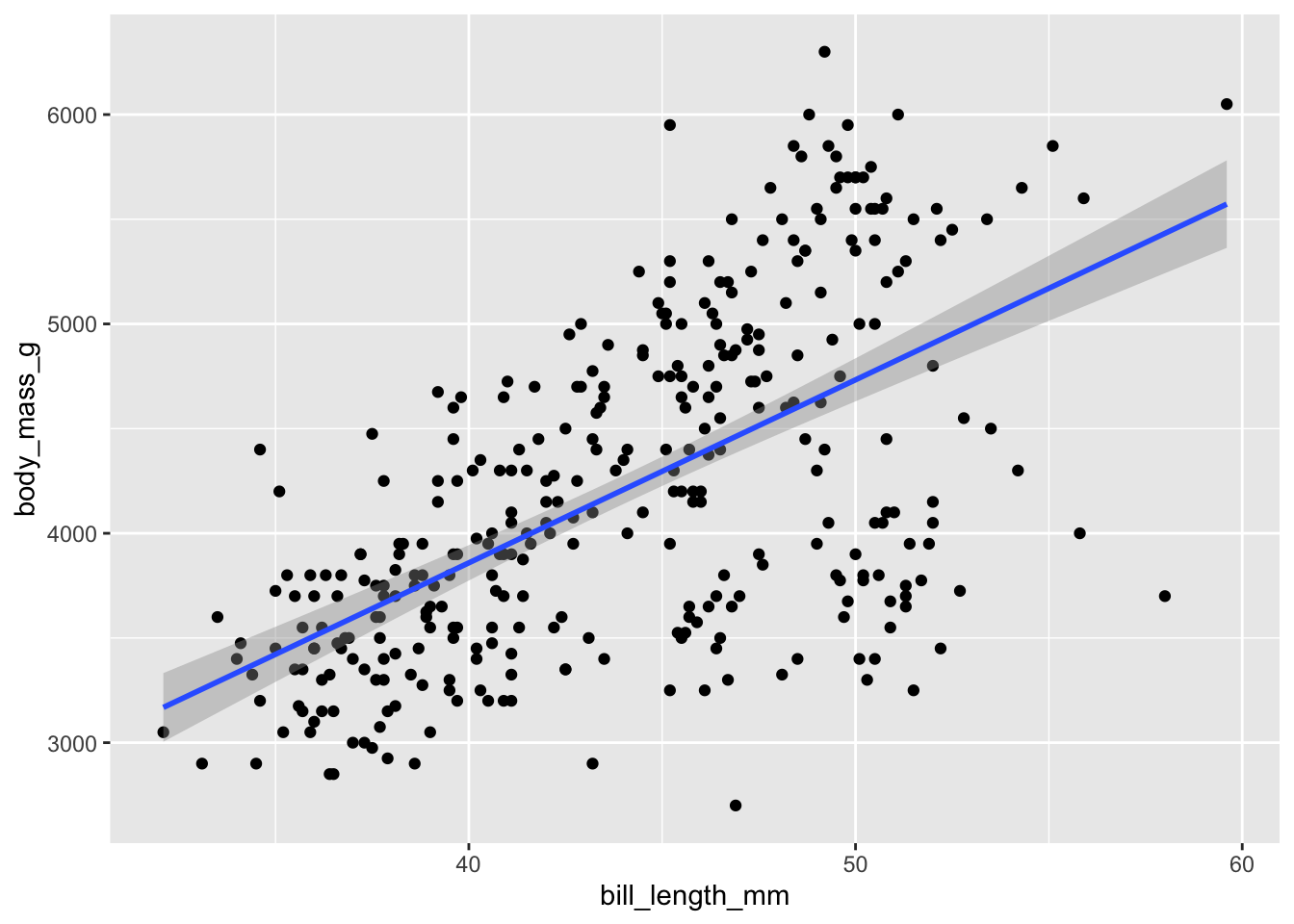### ANOVA Within a single species, are the body masses dependent on island? Only Adelie penguins were observed on all three islands. Let’s do an ANOVA. model.adelie = aov(body_mass_g ~ island, penguins %>% filter(species == "Adelie")) model.adelie Call: aov(formula = body_mass_g ~ island, data = penguins %>% filter(species == "Adelie")) Terms: island Residuals Sum of Squares 13655 31528779 Deg. of Freedom 2 148 Residual standard error: 461.5542 Estimated effects may be unbalanced 1 observation deleted due to missingness summary(model.adelie)  Df Sum Sq Mean Sq F value Pr(>F) island 2 13655 6827 0.032 0.968 Residuals 148 31528779 213032 1 observation deleted due to missingness anova(model.adelie) Analysis of Variance Table Response: body_mass_g Df Sum Sq Mean Sq F value Pr(>F) island 2 13655 6827 0.032 0.9685 Residuals 148 31528779 213032  How about the three different species, do they have different bill sizes? model.bill = aov(bill_length_mm ~ species, data=penguins) model.bill Call: aov(formula = bill_length_mm ~ species, data = penguins) Terms: species Residuals Sum of Squares 7194.317 2969.888 Deg. of Freedom 2 339 Residual standard error: 2.959853 Estimated effects may be unbalanced 2 observations deleted due to missingness summary(model.bill)  Df Sum Sq Mean Sq F value Pr(>F) species 2 7194 3597 410.6 <2e-16 *** Residuals 339 2970 9 --- Signif. codes: 0 '***' 0.001 '**' 0.01 '*' 0.05 '.' 0.1 ' ' 1 2 observations deleted due to missingness anova(model.bill) Analysis of Variance Table Response: bill_length_mm Df Sum Sq Mean Sq F value Pr(>F) species 2 7194.3 3597.2 410.6 < 2.2e-16 *** Residuals 339 2969.9 8.8 --- Signif. codes: 0 '***' 0.001 '**' 0.01 '*' 0.05 '.' 0.1 ' ' 1 # Chi-square and Categorical Data Chi-square testing in SciPy is unfortunately almost as much work as doing the entire test by hand. There is the function scipy.stats.chisquare, but it requires you to input the expected frequencies (unless they are all equal) and an adjustement value to the degrees of freedom (if it is anything other than $$k-1$$). As a result, this test works comfortably for testing Goodness of Fit against equally likely categories, but in very few other situations. ### Uniform Distribution Goodness of Fit The homework question 13.4 asks whether 120 released homing pigeons indicate a preference in flight direction. The data given is: from tabulate import tabulate pigeons = [12, 16, 17, 15, 13, 20, 17, 10] print(tabulate([pigeons]))  12 16 17 15 13 20 17 10 Since the question is asking for equal frequencies, the scipy.stats.chisquare function is easy to use: scipy.stats.chisquare(pigeons) Power_divergenceResult(statistic=4.8, pvalue=0.6843549385011479) ### Goodness of Fit Exercise 13.6 asks about the distribution of sorghum cultivars and their seed colors. From genetic theory, red/yellow/white should occur in the ratios 9:3:4. An experiment has counted 195 red, 73 yellow and 100 white. Does this data confirm or contradict the theory? This exercise calls for a little bit more work on using scipy.stats.chisquare: we need to provide expected counts. So we sum up the total number, figure out the proportions indicated by the given ratios, and multiply them together. Since no quantities were estimated, we do not need to adjust the degrees of freedom here. import numpy as np # data given ratios = np.array([9, 3, 4]) observed = np.array([195, 73, 100]) # computed data props = ratios/ratios.sum() expected = observed.sum()*props print(tabulate([props])) ------ ------ ---- 0.5625 0.1875 0.25 ------ ------ ---- print(tabulate([expected])) --- -- -- 207 69 92 --- -- -- scipy.stats.chisquare(observed, expected) Power_divergenceResult(statistic=1.6231884057971016, pvalue=0.444149437203624) ### Independence of rows and columns in a contingency table For independence tests in contingency tables, the module scipy.stats.contingency has several useful functions available: chi2_contingency for the chi-square test, and crosstab to create two-way tables from observations. We might, for instance, ask whether the Palmer penguins’ species and island assignments are independent variables:  penguins_levels, penguins_xt = scipy.stats.contingency.crosstab(penguins.species.to_list(), penguins.island.to_list()) print(tabulate(penguins_xt)) --- -- -- 44 55 47 0 68 0 119 0 0 --- -- -- penguins_x2, penguins_p, penguins_dof, penguins_Eij = scipy.stats.contingency.chi2_contingency(penguins_xt) print(f"X^2 statistic: {penguins_x2:.2f}, {penguins_dof} Degrees of Freedom yield a p-value of {penguins_p:.2e}") X^2 statistic: 284.59, 4 Degrees of Freedom yield a p-value of 2.28e-60 Or if we have just the summary table in front of us (and thus don’t have to cross-tabulate ourselves): Exercise 13.27 Data is collected on frequencies of audiogenic seizures under different treatments with injections: import pandas as pd mice = pd.DataFrame({ "No Response": [21, 15, 23, 47], "Wild Running": [7, 14, 10, 13], "Clonic Seizure": [24, 20, 23, 28], "Tonic Seizure": [44, 54, 48, 32] }, index=["Thienylalanine","Solvent","Sham","Unhandled"], columns=["No Response", "Wild Running", "Clonic Seizure", "Tonic Seizure"]) print(tabulate(mice, headers="keys"))  No Response Wild Running Clonic Seizure Tonic Seizure -------------- ------------- -------------- ---------------- --------------- Thienylalanine 21 7 24 44 Solvent 15 14 20 54 Sham 23 10 23 48 Unhandled 47 13 28 32 We can simply feed this table straight into scipy.stats.contingency.chi2_contingency: stat, p, df, Eij = scipy.stats.contingency.chi2_contingency(mice) print(f"X^2 statistic: {stat:.2f}, {df} Degrees of Freedom yield a p-value of {p:.2e}") X^2 statistic: 27.66, 9 Degrees of Freedom yield a p-value of 1.09e-03 In R, the function chisq.test does a lot of work to figure out what kind of a chi-square test you might be trying to do, and to do the computations for you as far as possible. ### Uniform Distribution Goodness of Fit The homework question 13.4 asks whether 120 released homing pigeons indicate a preference in flight direction. The data given is: pigeons = c(12, 16, 17, 15, 13, 20, 17, 10) pigeons %>% t %>% kable  12 16 17 15 13 20 17 10 Just giving the frequencies as-is to chisq.test will make R assume that you want a uniform goodness of fit test, and perform just that. chisq.test(pigeons)  Chi-squared test for given probabilities data: pigeons X-squared = 4.8, df = 7, p-value = 0.6844 ### Goodness of Fit Exercise 13.6 asks about the distribution of sorghum cultivars and their seed colors. From genetic theory, red/yellow/white should occur in the ratios 9:3:4. An experiment has counted 195 red, 73 yellow and 100 white. Does this data confirm or contradict the theory? By additionally giving the p argument with a sequence of probabilities, or adding rescale.p=TRUE to make R rescale to probabilities for you, you can make a goodness of fit test against any other (finite) distribution. ratios = c(9,3,4) observed = c(195, 73, 100) chisq.test(observed, p=ratios, rescale.p=TRUE)  Chi-squared test for given probabilities data: observed X-squared = 1.6232, df = 2, p-value = 0.4441 ### Independence of rows and columns in a contingency table R makes it easy to create contingency tables using either the table command if you have a matrix of frequencies, or xtab if you have a dataframe with key pairs and frequencies. We might, for instance, ask whether the Palmer penguins’ species and island assignments are independent variables. In R, you can perform this test by simply giving both a vector for x and for y, the two first positional arguments to chisq.test. It will then compute the contingency table for you and perform the independence chi-square test. Alternatively, if you have a contingency table already, you can feed it directly into chisq.test and it will do the same thing: perform an independence test. chisq.test(penguinsspecies, penguins$island)  Pearson's Chi-squared test data: penguins$species and penguins\$island
X-squared = 299.55, df = 4, p-value < 2.2e-16

Or, using the xtabs function to count co-occurrences for us:

penguins.xt = xtabs(~species+island, data=penguins)
penguins.xt %>% kable
Biscoe Dream Torgersen
Chinstrap 0 68 0
Gentoo 124 0 0
chisq.test(penguins.xt)

Pearson's Chi-squared test

data:  penguins.xt
X-squared = 299.55, df = 4, p-value < 2.2e-16

Or, if we are given an explicit contingency table with frequencies, such as in Exercise 13.27:

Exercise 13.27 Data is collected on frequencies of audiogenic seizures under different treatments with injections:

mice = tribble(
~Treatment, ~NoResponse, ~WildRunning, ~Clonic, ~Tonic,
"Thienylalanine", 21, 7, 24, 44,
"Solvent", 15, 14, 20, 54,
"Sham", 23, 10, 23, 48,
"Unhandled", 47, 13, 28, 32
)
mice.df = mice %>% pivot_longer(cols=-Treatment)
mice.xt = xtabs(value ~ Treatment+name, mice.df)
mice.xt %>% kable
Clonic NoResponse Tonic WildRunning
Sham 23 23 48 10
Solvent 20 15 54 14
Thienylalanine 24 21 44 7
Unhandled 28 47 32 13

By handing a matrix to chisq.test, it assumes this is a contingency table and tests for independence.

chisq.test(mice.xt)

Pearson's Chi-squared test

data:  mice.xt
X-squared = 27.664, df = 9, p-value = 0.001085

# Some important plot types

In Python, the standard plotting package is matplotlib, with several useful addon-packages and extensions - including seaborn and probscale. In matplotlib there is a comfortable set of commands to do almost everything you might need in the module pyplot.

For seaborn plot commands, give a pandas DataFrame as the data argument, and give x, y as the strings corresponding to the column names. You can also usually give hue for colors, and sometimes size and style - in each case the system will take the data in the corresponding variable and encode it as either x-position, y-position, color, size or style of the thing you draw.

For the examples in this section, we will be using the auto-mpg dataset (included among other places in scikit-learn, the main machine learning library for Python)

print(mpg.head(5).to_markdown())
mpg cylinders displacement horsepower weight acceleration model year origin car name
0 18 8 307 130 3504 12 70 1 chevrolet chevelle malibu
1 15 8 350 165 3693 11.5 70 1 buick skylark 320
2 18 8 318 150 3436 11 70 1 plymouth satellite
3 16 8 304 150 3433 12 70 1 amc rebel sst
4 17 8 302 140 3449 10.5 70 1 ford torino

### Single variable (categorical)

To visualize the distribution of a single categorical variable, we usually end up using some version of a bar diagram.

from matplotlib import pyplot
import seaborn

pyplot.figure()
seaborn.countplot(x="cylinders", data=mpg)
pyplot.show()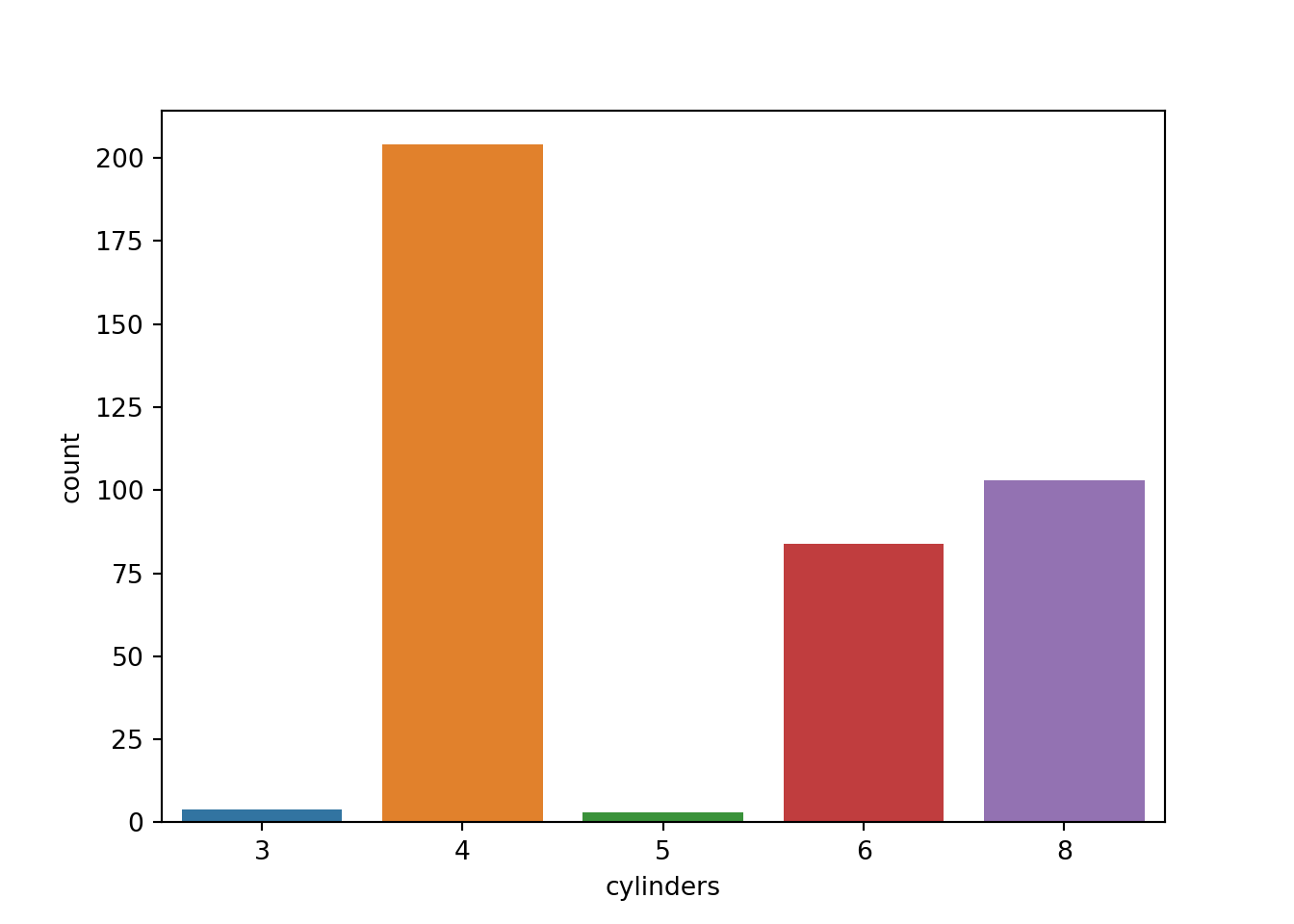### Single variable (continuous)

For a single continuous variable, density plots or histograms are often used to show their distributions:

# Try also stats
# - "count"       - number in each bin
# - "frequency"   - number / bin-width
# - "probability" - bar-heights sum to 1
# - "density"     - total area sums to 1
pyplot.figure()
seaborn.histplot(x="horsepower", data=mpg, bins=20, stat="probability")
pyplot.title("Histogram of Horsepower")
pyplot.show()
pyplot.figure()
seaborn.kdeplot(x="mpg", data=mpg)
pyplot.title("Density plot of MPG")
pyplot.show()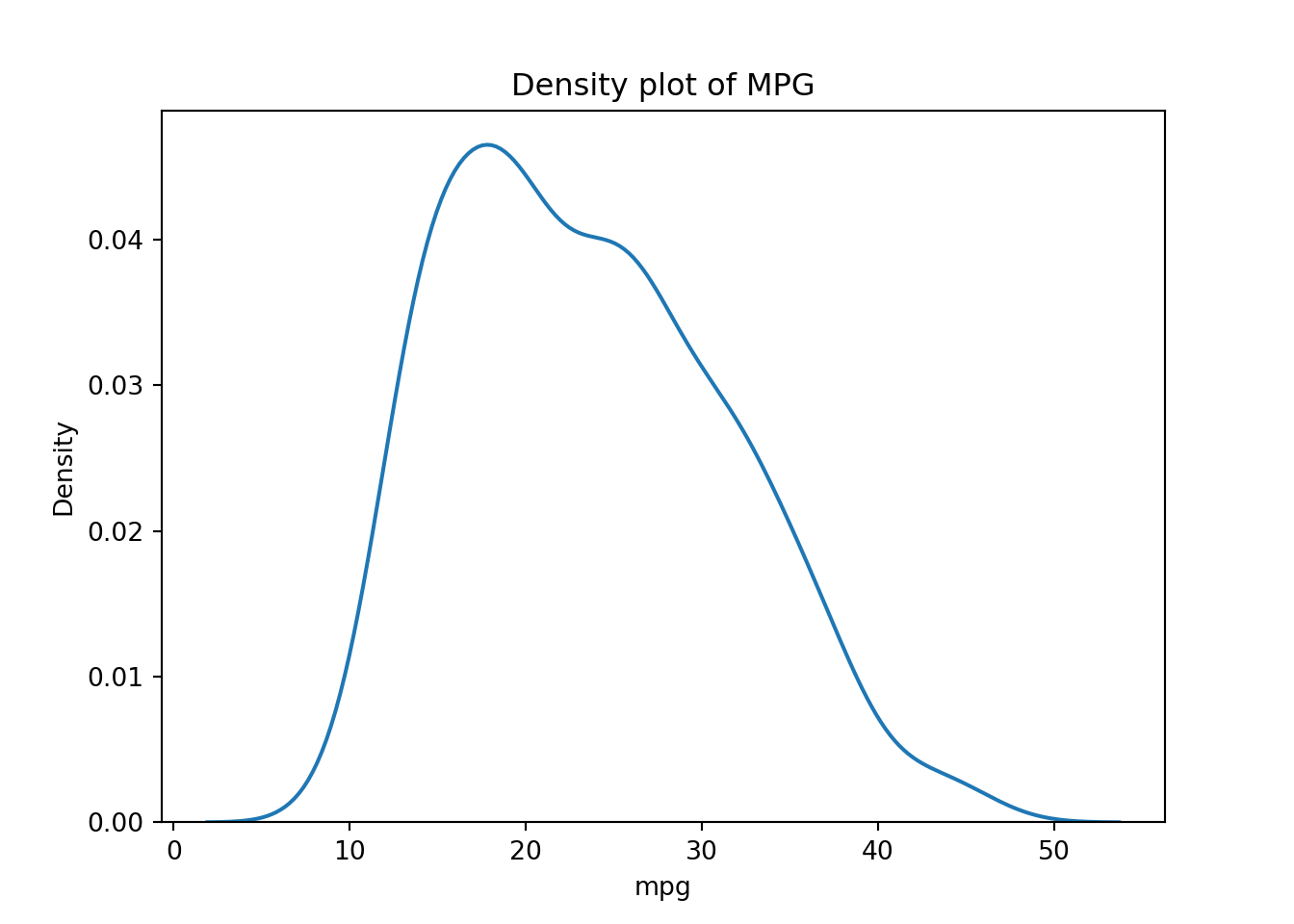### Continuous vs. continuous

The gold standard for visualizing the relationship for paired continuous variables is the scatter plot.

pyplot.figure()
seaborn.scatterplot(x="horsepower",y="mpg",data=mpg)
pyplot.show()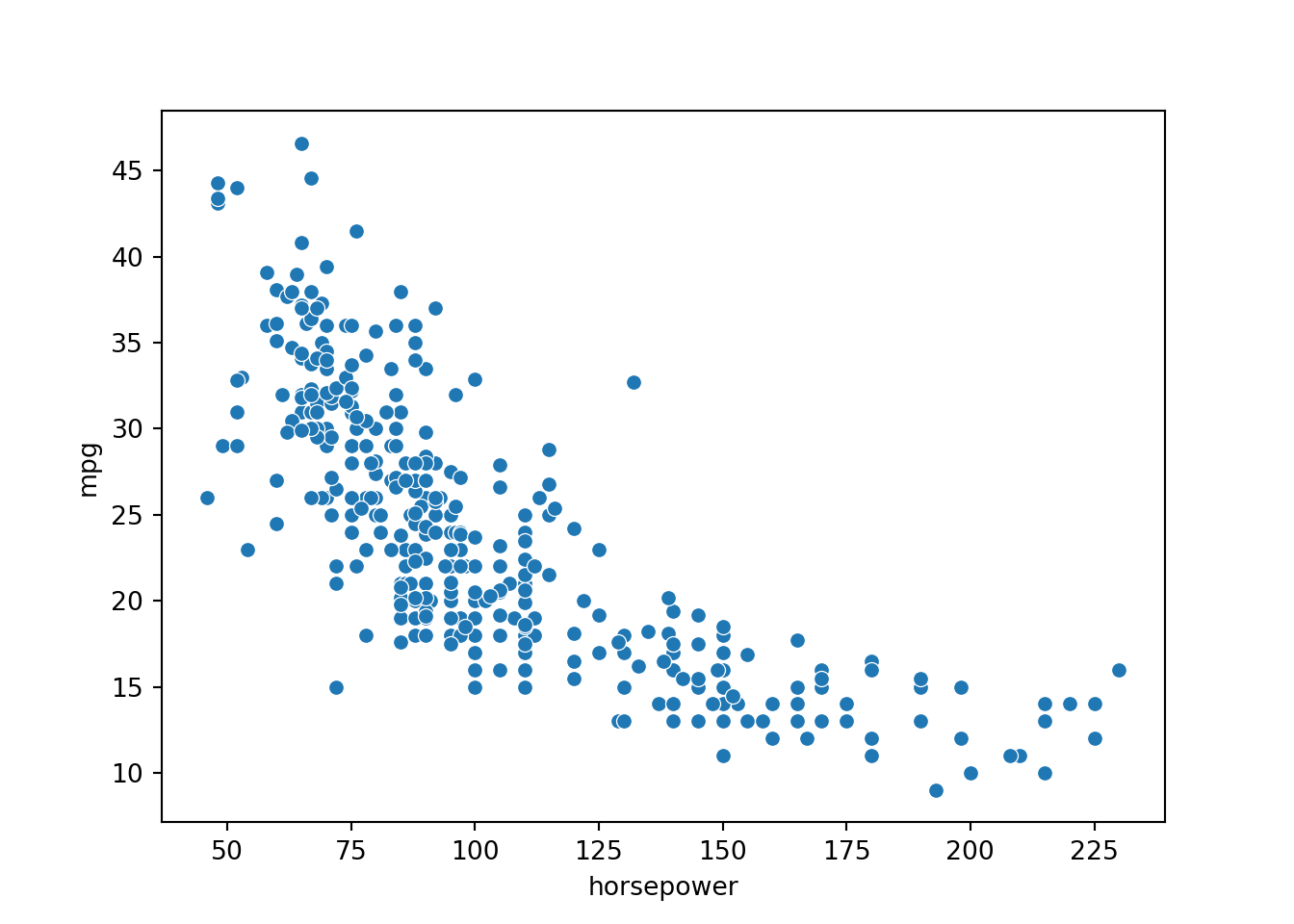#### Many variables at once

pyplot.figure()
seaborn.scatterplot(x="horsepower",
y="mpg",
hue="cylinders",
size="weight",
style="origin",
data=mpg)
pyplot.show()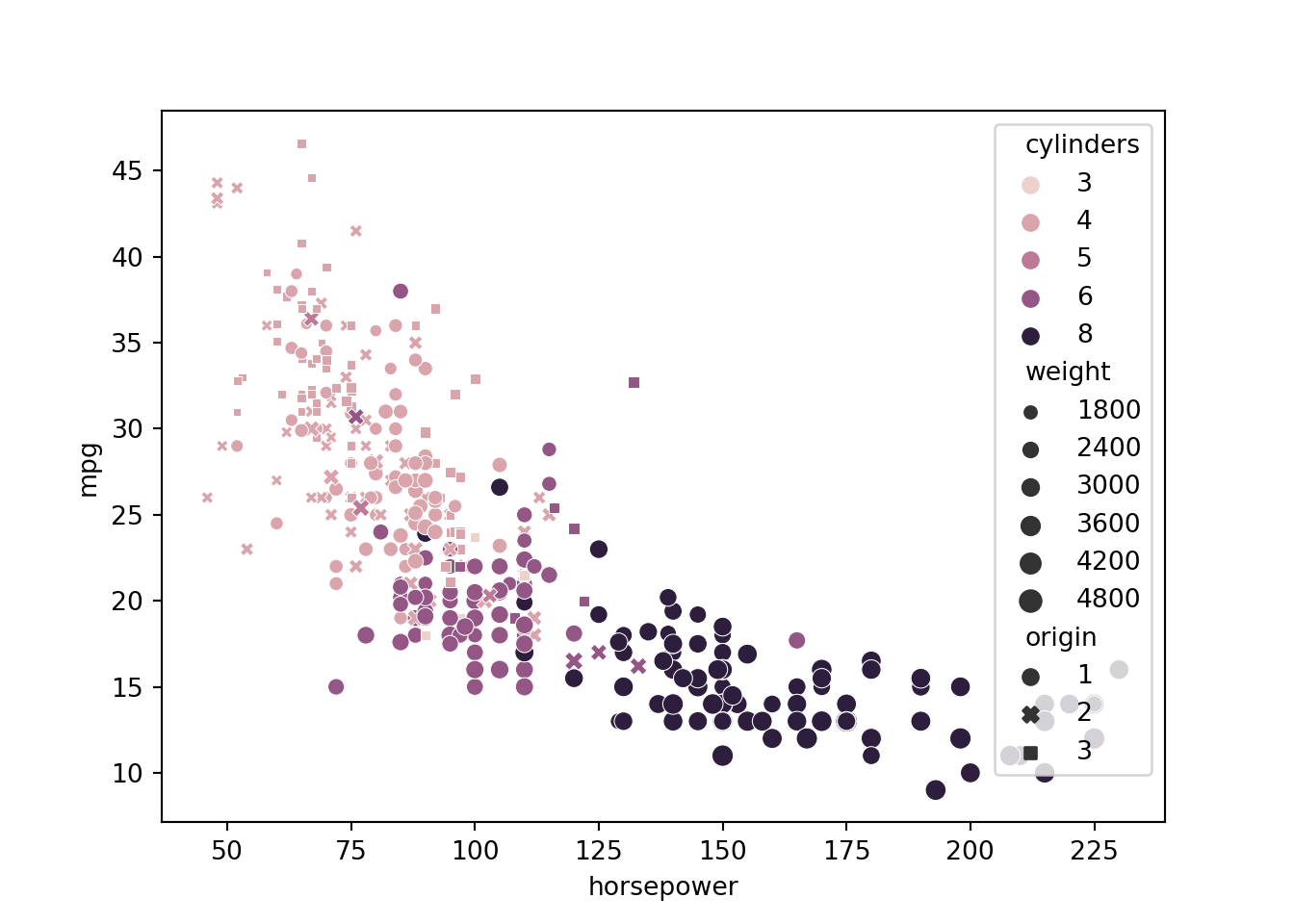### Continuous vs. categorical

The interactions between continuous and categorical variables is most often shown with side-by-side distribution plots of different kinds. Some very useful ones include the boxplot, the violinplot, density plots, strip plots and swarm plots. Most of these can be accessed through the seaborn.catplot function for plotting categorical data.

pyplot.figure(figsize=(4,6));
seaborn.catplot(x="cylinders",y="horsepower",data=mpg);
pyplot.title("Strip plot (default) of cylinders vs. horsepower");
pyplot.show()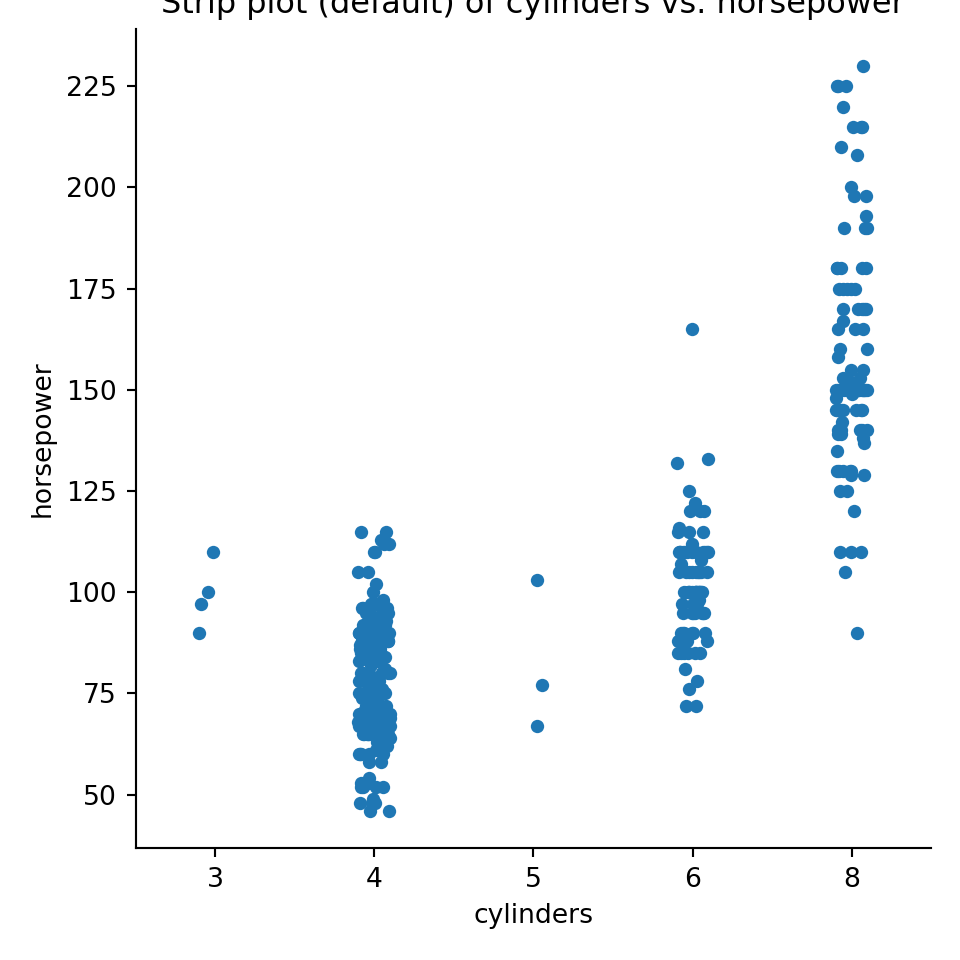pyplot.figure(figsize=(4,6));
seaborn.catplot(x="cylinders",y="horsepower",data=mpg, kind="swarm");
pyplot.title("Swarm plot of cylinders vs. horsepower");
pyplot.show()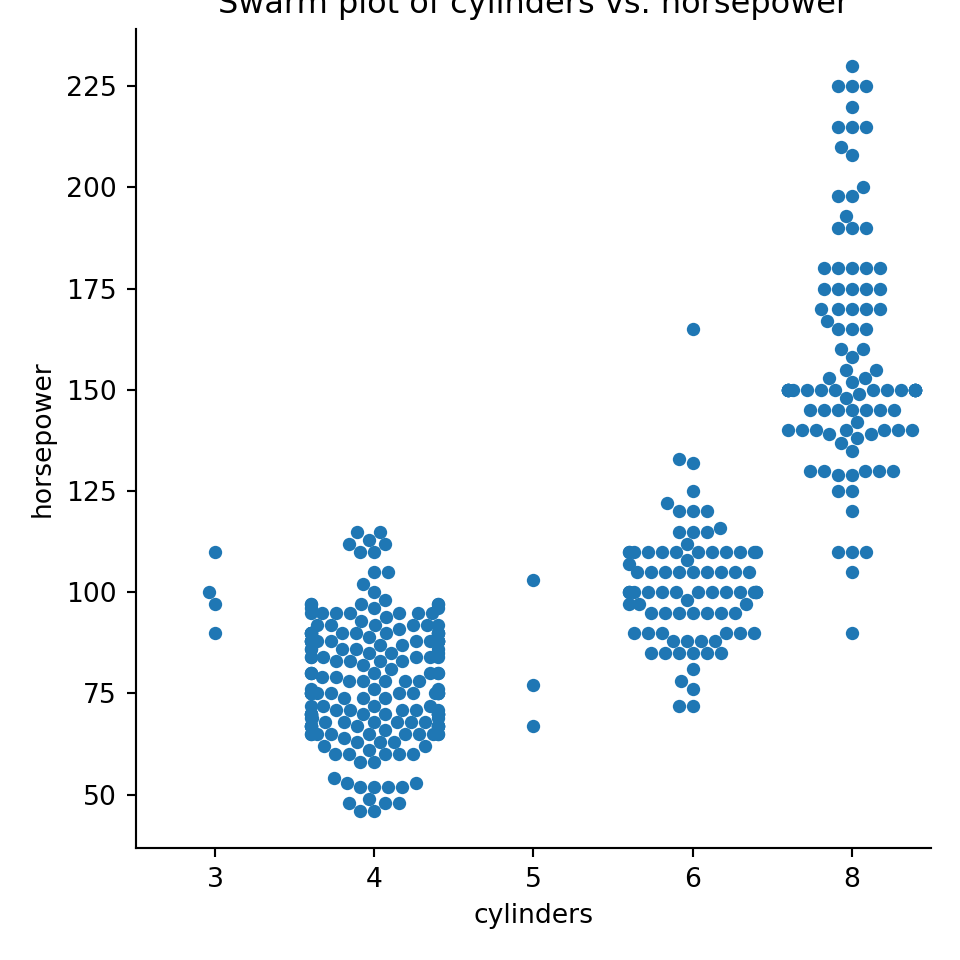pyplot.figure(figsize=(4,6));
seaborn.catplot(x="cylinders",y="horsepower",data=mpg, kind="box");
pyplot.title("Box plot of cylinders vs. horsepower");
pyplot.show()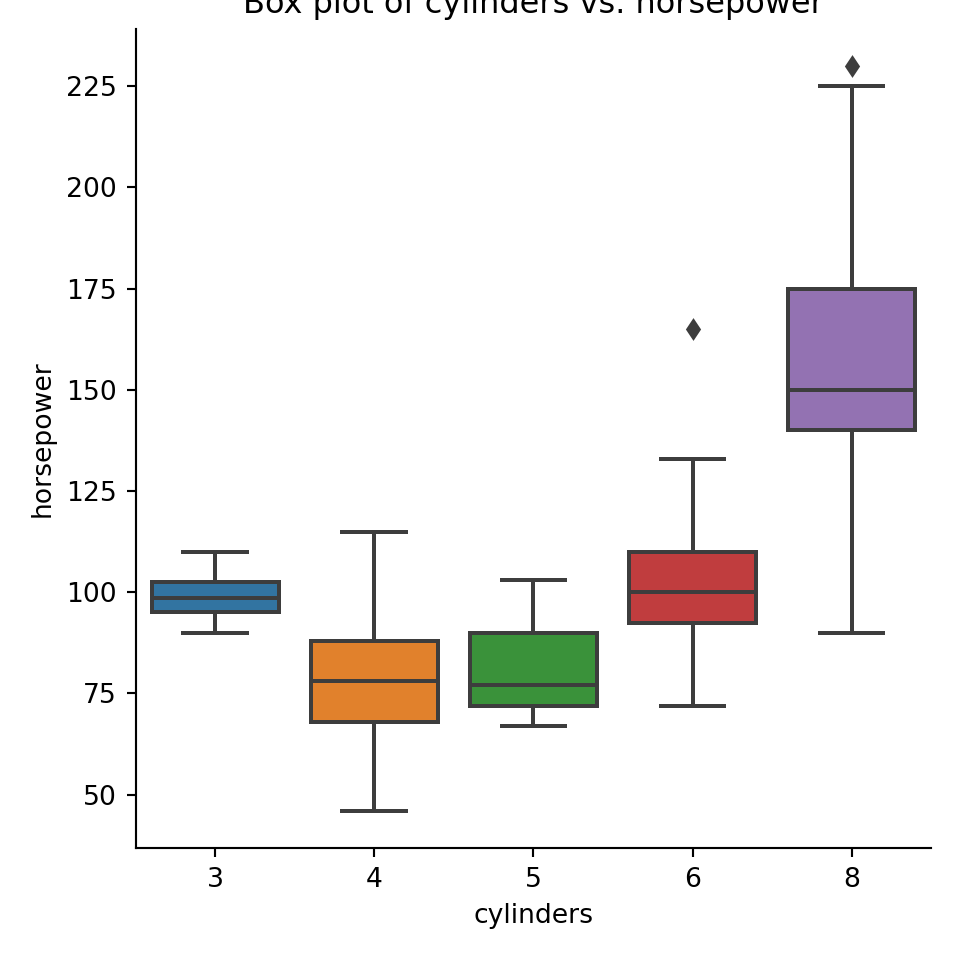pyplot.figure(figsize=(4,6));
seaborn.catplot(x="cylinders",y="horsepower",data=mpg, kind="boxen");
pyplot.title("Enhanced box plot of cylinders vs. horsepower");
pyplot.show()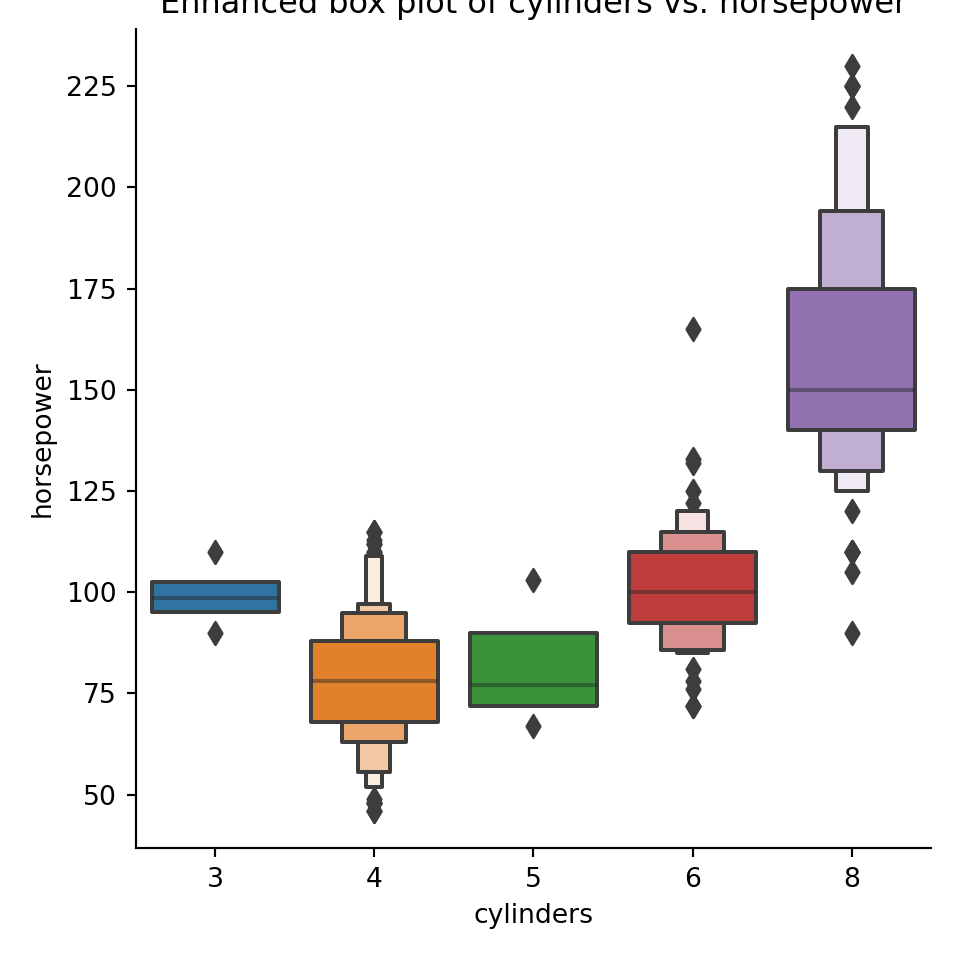pyplot.figure(figsize=(4,6));
seaborn.catplot(x="cylinders",y="horsepower",data=mpg, kind="violin", ax=pyplot.gca());
pyplot.title("Violin plot of cylinders vs. horsepower");
pyplot.show()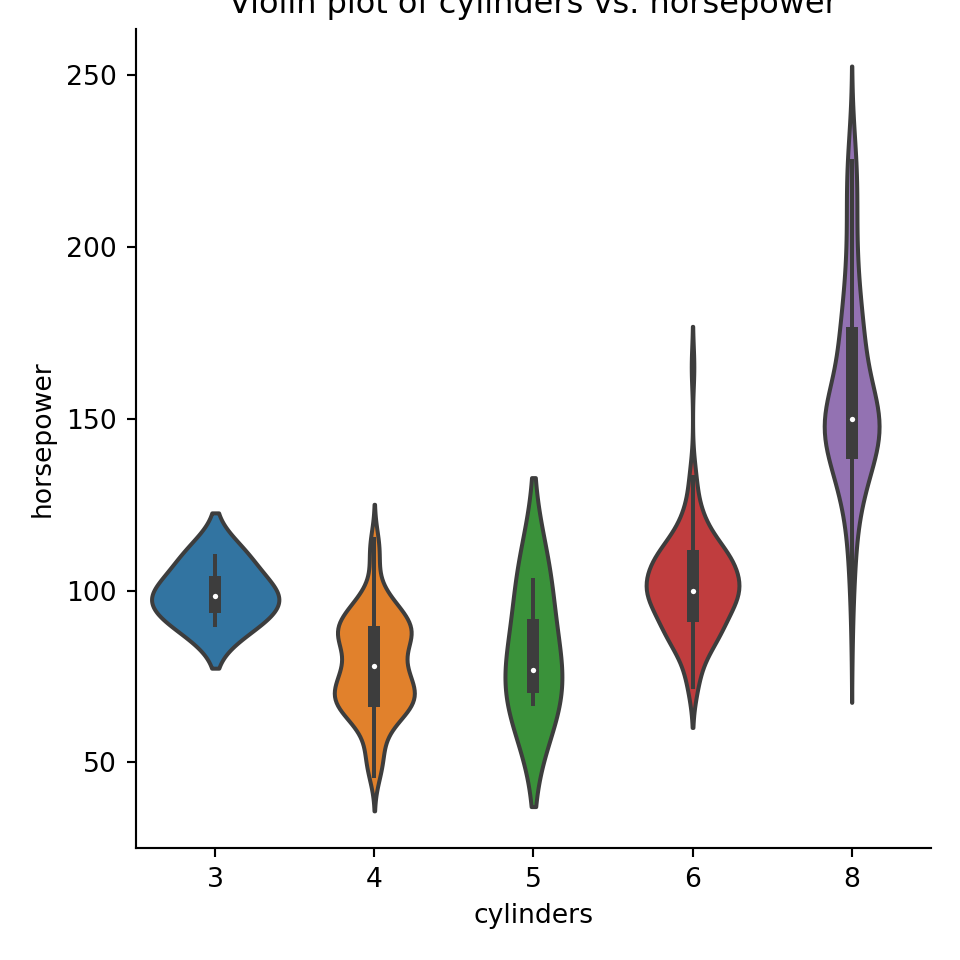pyplot.figure(figsize=(4,6));
seaborn.displot(hue="cylinders",x="horsepower",data=mpg, kind="kde", rug=True);
pyplot.title("Density and rug plots of horsepower, colored by cylinder count");
pyplot.show()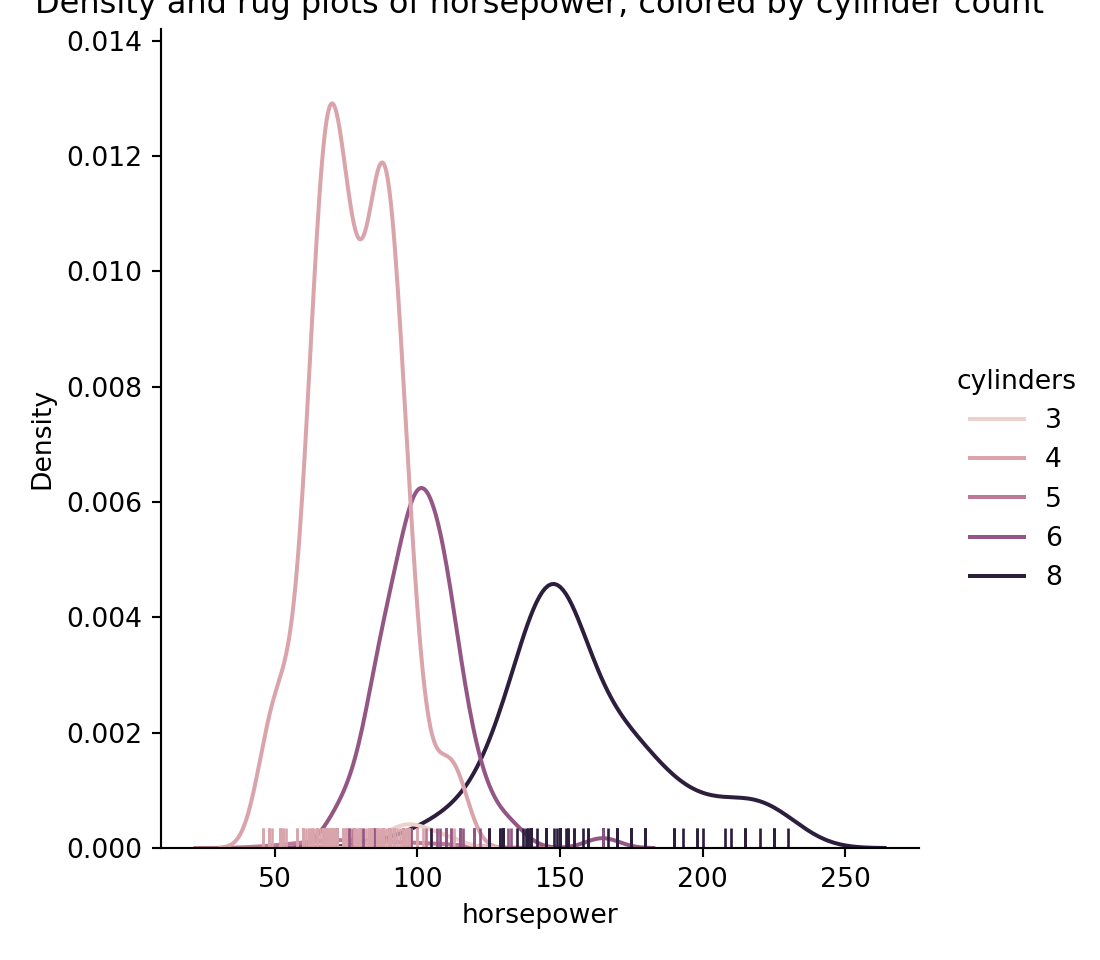### Categorical vs. categorical

For these kinds of plots, the most common choice is for something like a dodged bar plot or a stacked bar plot. Something like this:

import seaborn.objects as so

pyplot.figure()
seaborn.catplot(x="cylinders", hue="origin", data=mpg, kind="count")
pyplot.title("Dodged bar chart")
pyplot.show()
pyplot.figure()
so.Bar(), so.Hist(), so.Stack()
).on(pyplot.gcf()).plot()
pyplot.title("Dodged bar chart")
pyplot.show()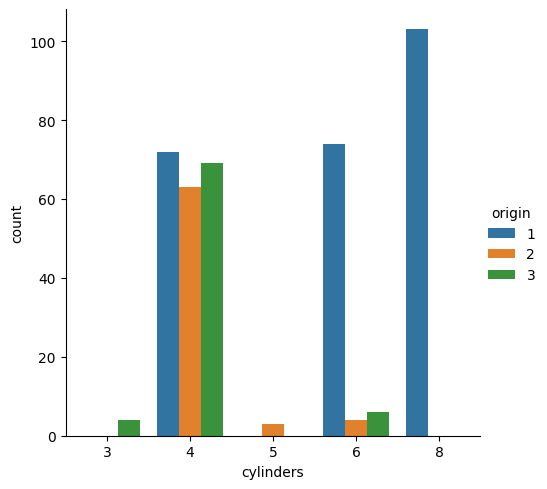<seaborn._core.plot.Plotter object at 0x29a075e10>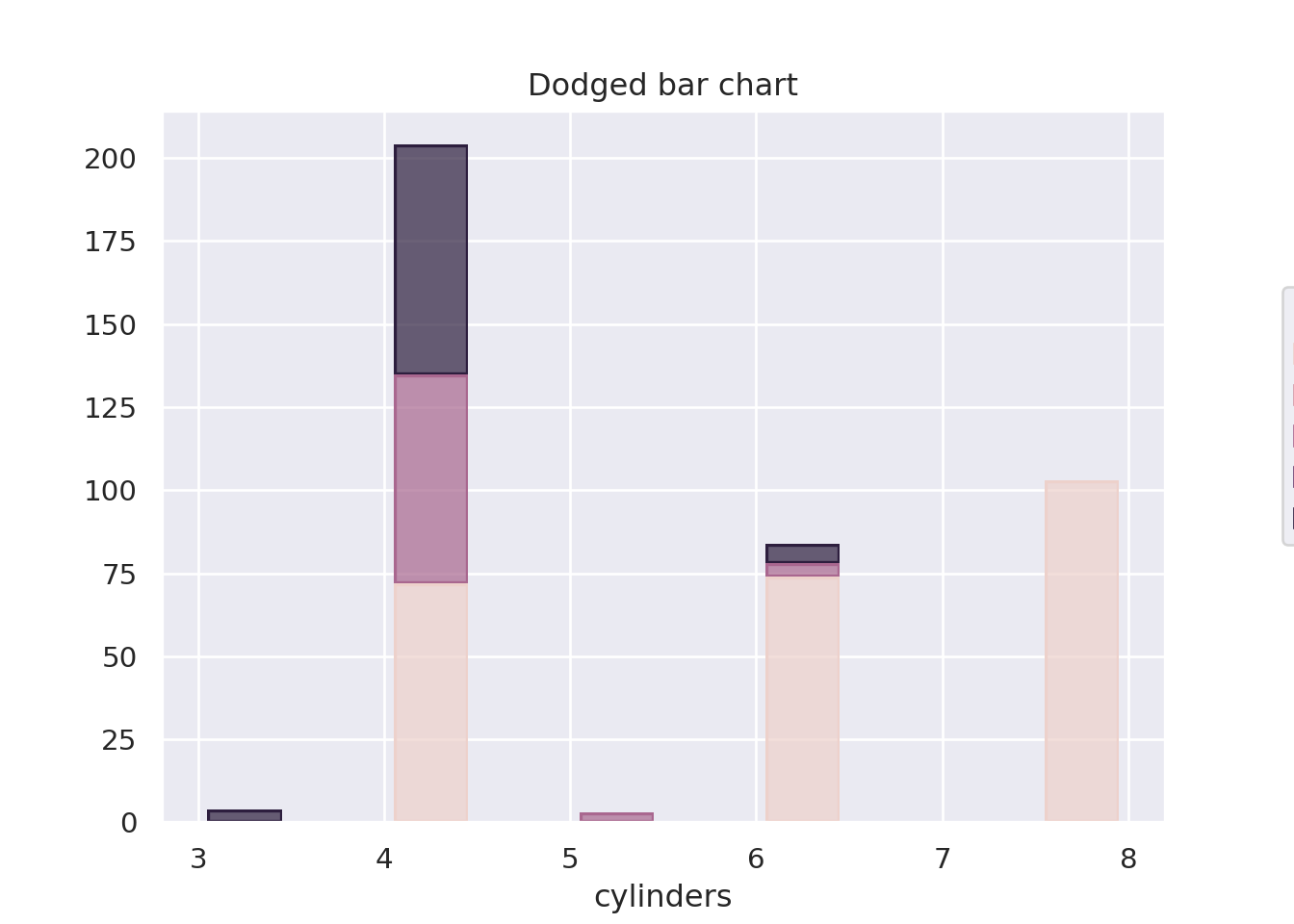### Checking assumptions

The most common assumption we need to check with appropriate plots is the normality assumption - we do this with a probability plot or a quantile plot.

scipy.stats.probplot does the job for us, as does probscale.probplot:

import scipy, scipy.stats

f,ax = pyplot.subplots()
scipy.stats.probplot(mpg.acceleration, plot=ax);
pyplot.show()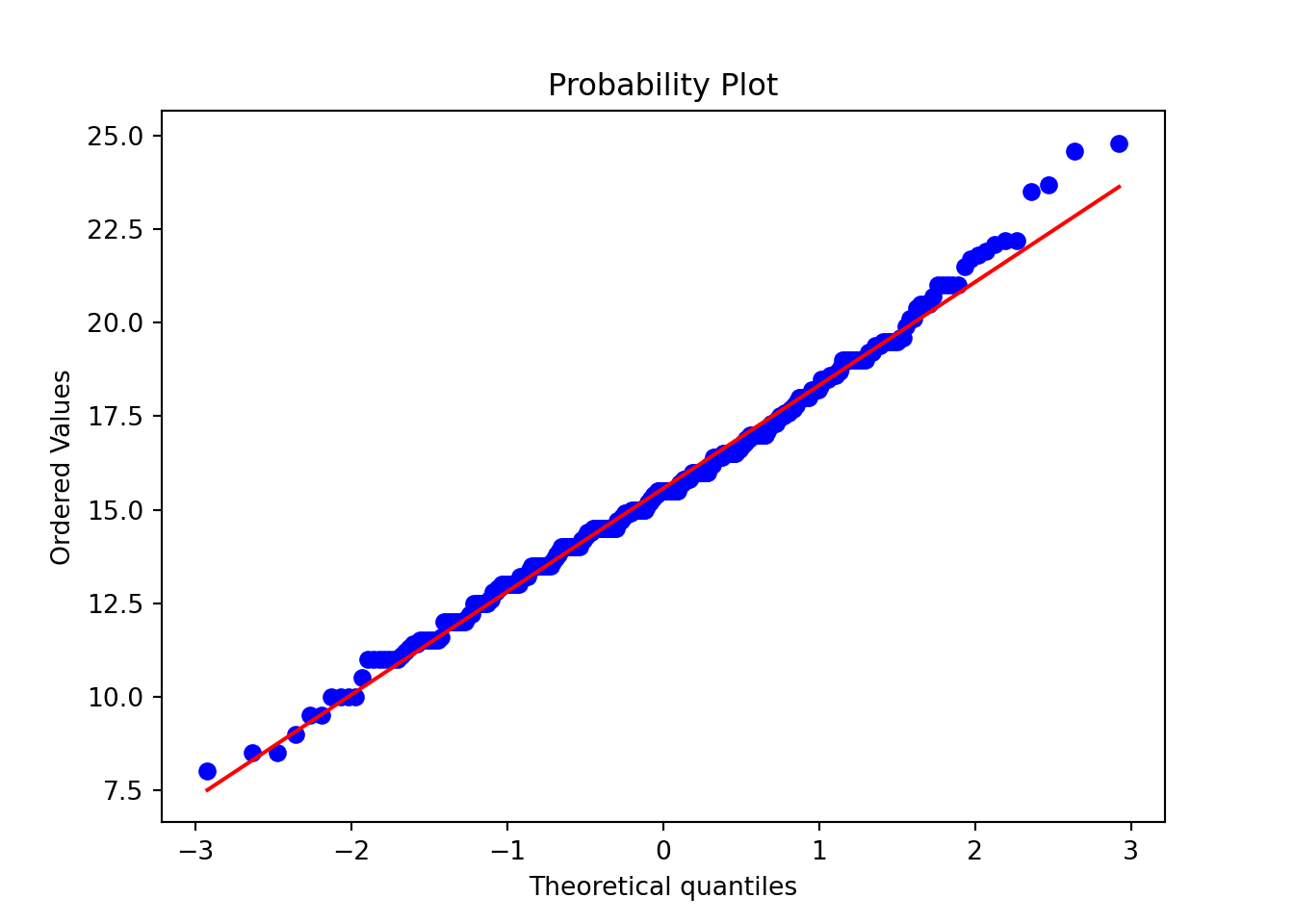import probscale

f,ax = pyplot.subplots()
probscale.probplot(mpg.acceleration, ax=ax);
pyplot.show()tidyverse includes my favorite plot package: ggplot2, implementing the Grammar of Graphics. A typical ggplot2 plot is made by:

1. Creating a plot canvas (with ggplot(df))
2. Specifying how data columns connect to visual attributes (with an aes(x,y,...) statement)
3. Picking what geometric marks to draw (with geom_-functions)
4. Fine-tuning the plot

We will use the mpg dataset from ggplot2 for these examples:

library(tidyverse)

theme_set(theme_light())
mpg %>% head(5) %>% kable()
manufacturer model displ year cyl trans drv cty hwy fl class
audi a4 1.8 1999 4 auto(l5) f 18 29 p compact
audi a4 1.8 1999 4 manual(m5) f 21 29 p compact
audi a4 2.0 2008 4 manual(m6) f 20 31 p compact
audi a4 2.0 2008 4 auto(av) f 21 30 p compact
audi a4 2.8 1999 6 auto(l5) f 16 26 p compact

### Single variable (categorical)

To visualize the distribution of a single categorical variable, we usually end up using some version of a bar diagram.

ggplot(mpg, aes(x=drv)) +
geom_bar() +
xlab("Drive type (front/rear/4-wd)")### Single variable (continuous)

For a single continuous variable, density plots or histograms are often used to show their distributions. Count (the default for histogram) counts how many observations are in each “bin”, density rescales everything to integrate to 1 (default for density):

ggplot(mpg, aes(x=displ)) +
geom_histogram(bins=20) +
xlab("Engine Displacement")
ggplot(mpg, aes(x=displ)) +
geom_density() +
xlab("Engine Displacement")
ggplot(mpg, aes(x=displ)) +
geom_histogram(bins=20, aes(y=after_stat(density))) +
xlab("Engine Displacement (density)")
ggplot(mpg, aes(x=displ)) +
geom_density(aes(y=after_stat(count))) +
xlab("Engine Displacement (count)")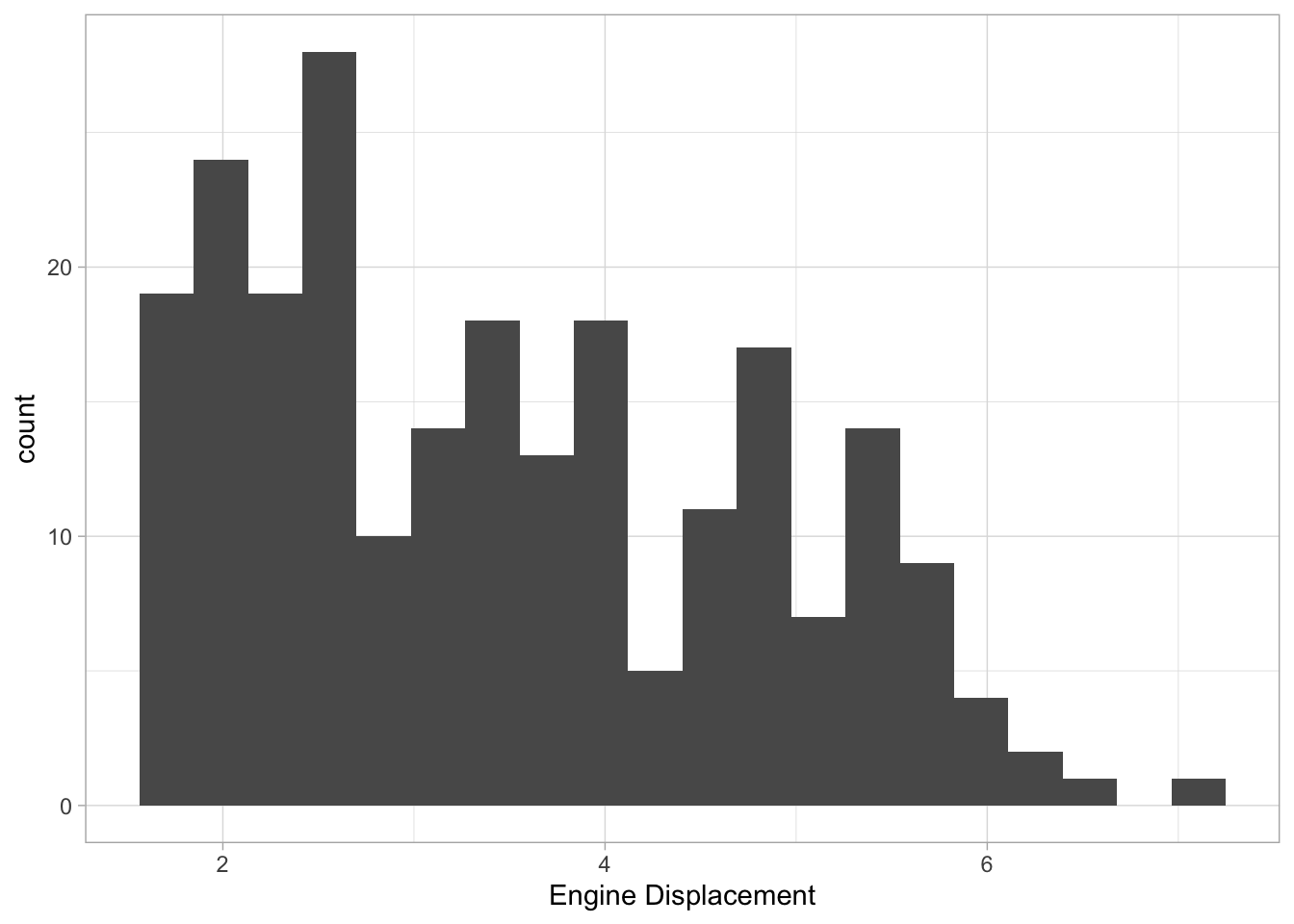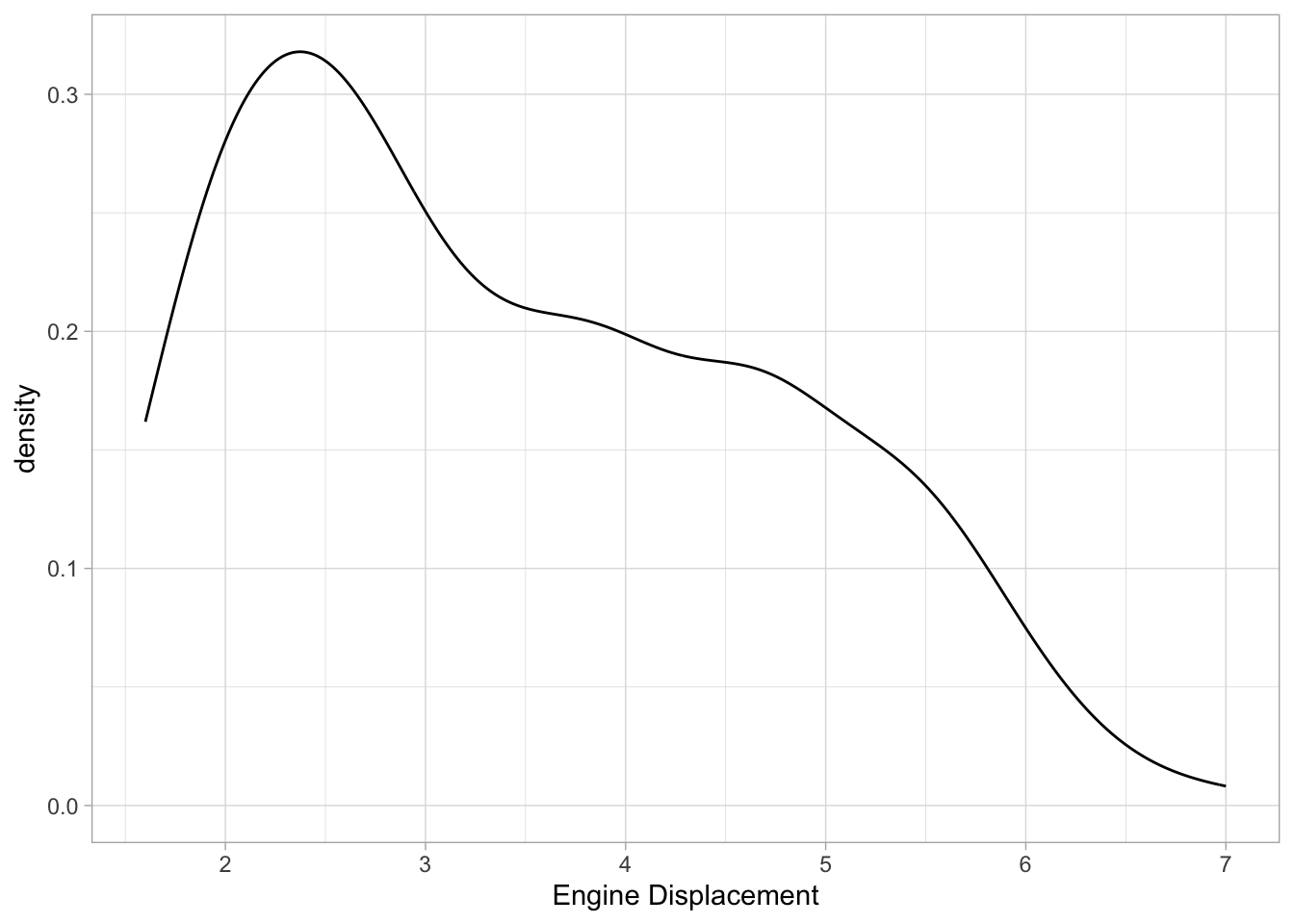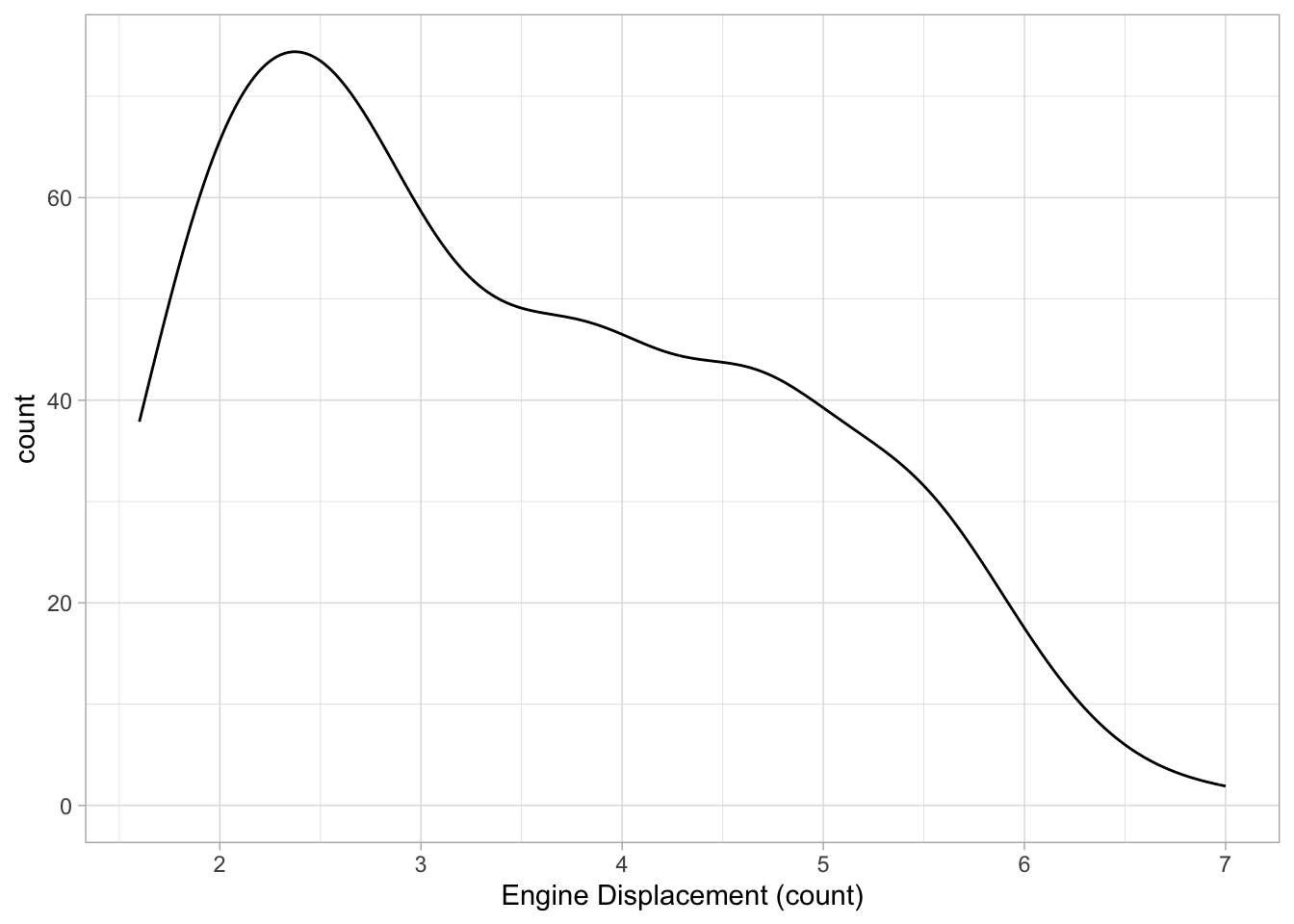### Continuous vs. continuous

The gold standard for visualizing the relationship for paired continuous variables is the scatter plot. In ggplot2, this is done with geom_point marks:

ggplot(mpg, aes(cty, hwy)) +
geom_point() +
labs(x="City MPG", y="Highway MPG")#### Many variables at once

You might find points stacking on top of each other. geom_jitter will move each point around a little bit to make sure they are all visible.

You can add more aesthetic mappings - such as color, style,

ggplot(mpg, aes(cty,hwy,shape=drv,color=class,size=displ)) +
geom_jitter() +
labs(x="City MPG", y="Highway MPG", shape="Drive type", size="Engine Displacement")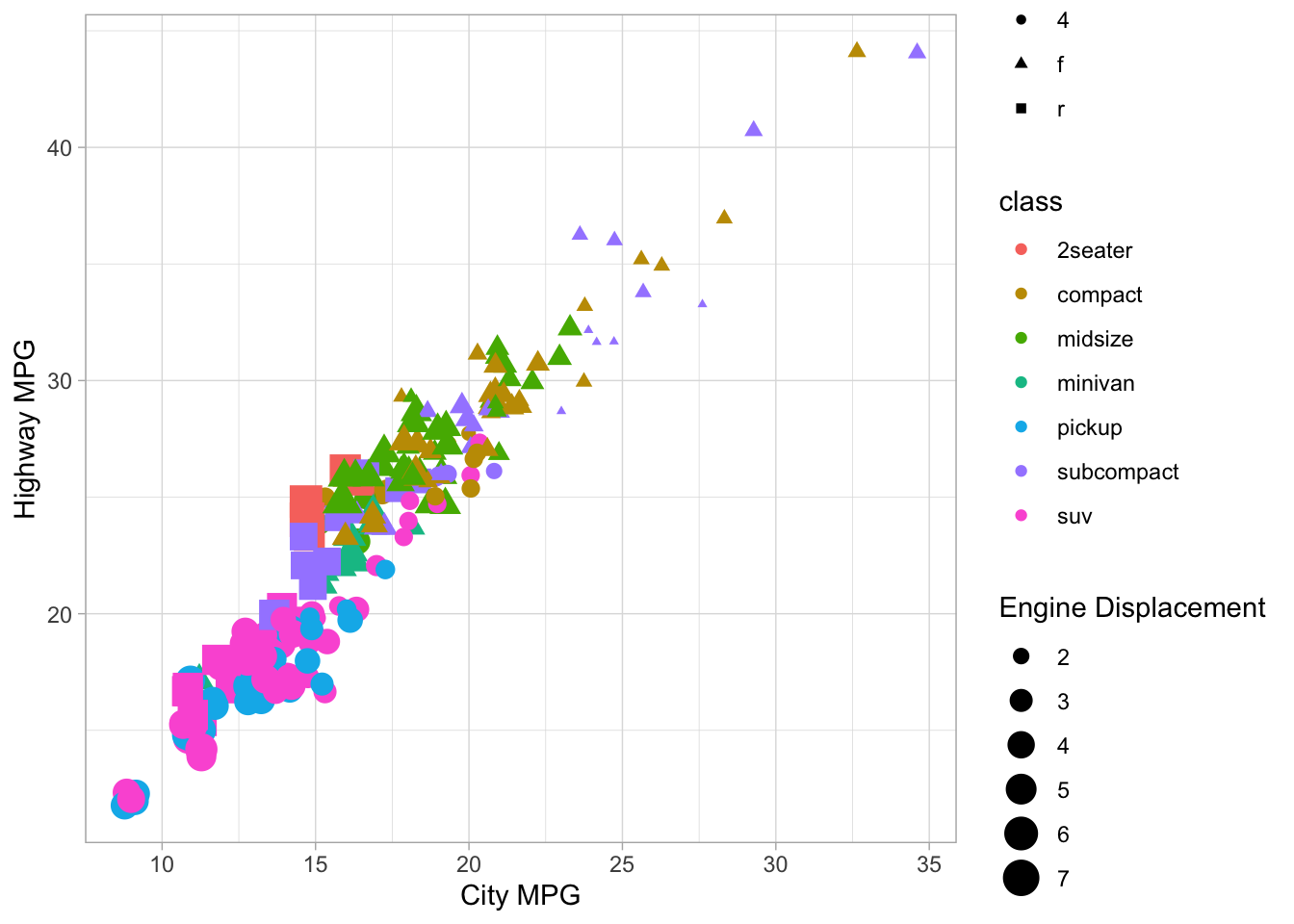### Continuous vs. categorical

The interactions between continuous and categorical variables is most often shown with side-by-side distribution plots of different kinds. Some very useful ones include the boxplot, the violinplot, density plots, strip plots and swarm plots.

library(ggbeeswarm)

ggplot(mpg, aes(x=drv, y=cty)) +
geom_boxplot() +
labs(x="Drive type", y="City MPG", title="Box plot")
ggplot(mpg, aes(x=drv, y=cty)) +
geom_violin() +
labs(x="Drive type", y="City MPG", title="Violin plot")
ggplot(mpg, aes(x=drv, y=cty)) +
geom_beeswarm() +
labs(x="Drive type", y="City MPG", title="Beeswarm plot")
ggplot(mpg, aes(color=drv, x=cty)) +
geom_density() +
geom_rug(aes(y=0), position=position_jitter(height=0, width=1.5)) +
labs(color="Drive type", x="City MPG", title="Simultaneous density plots")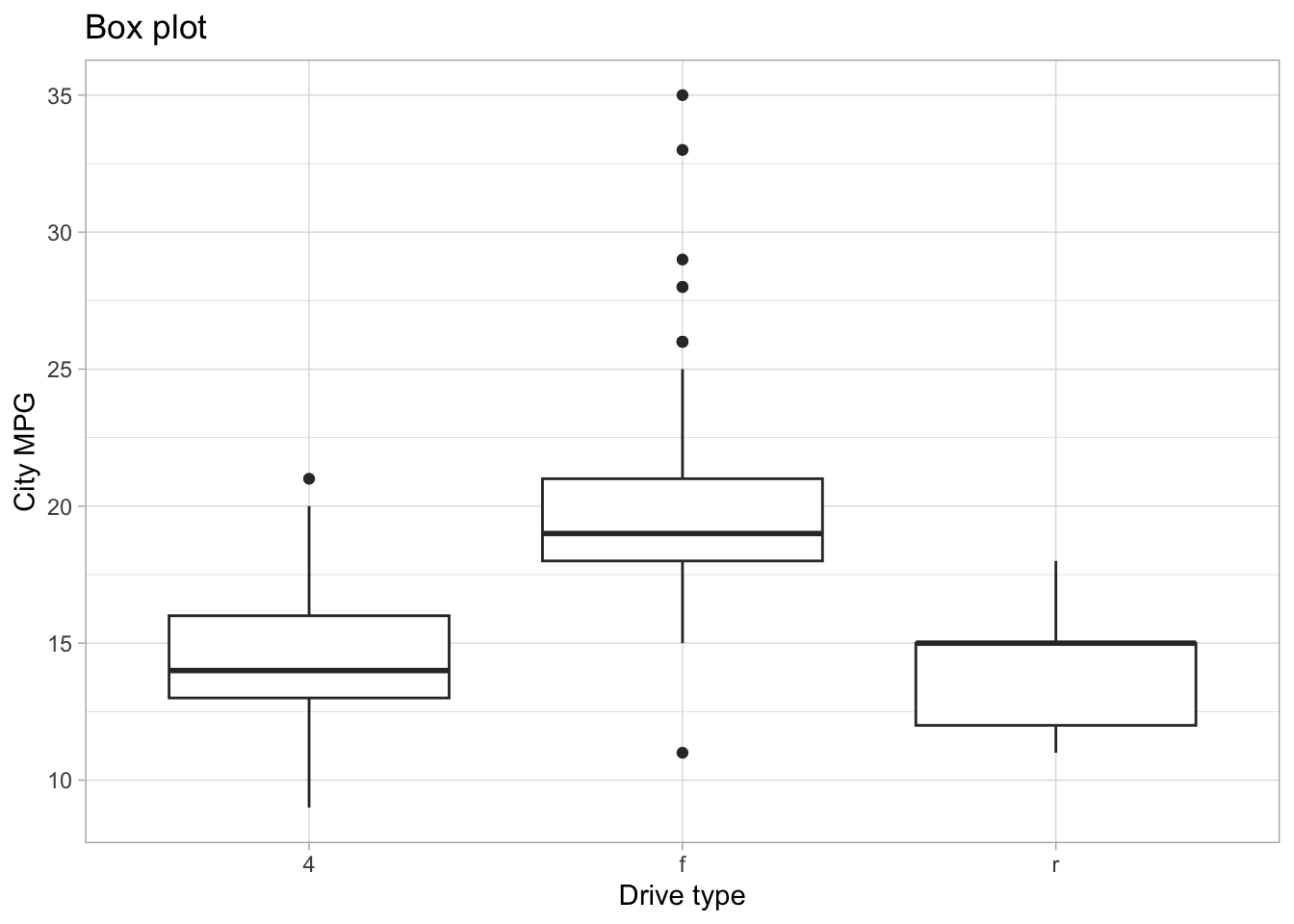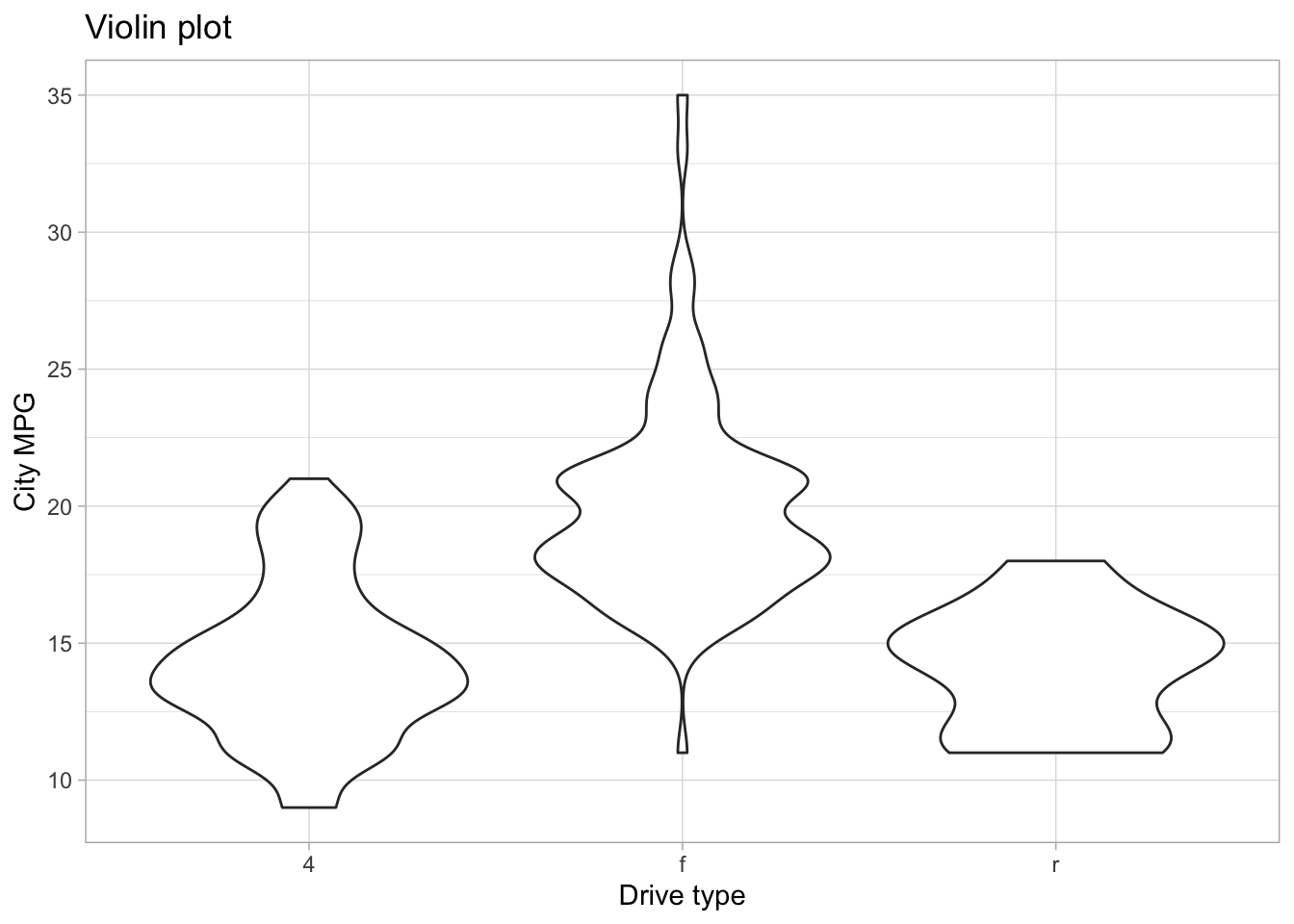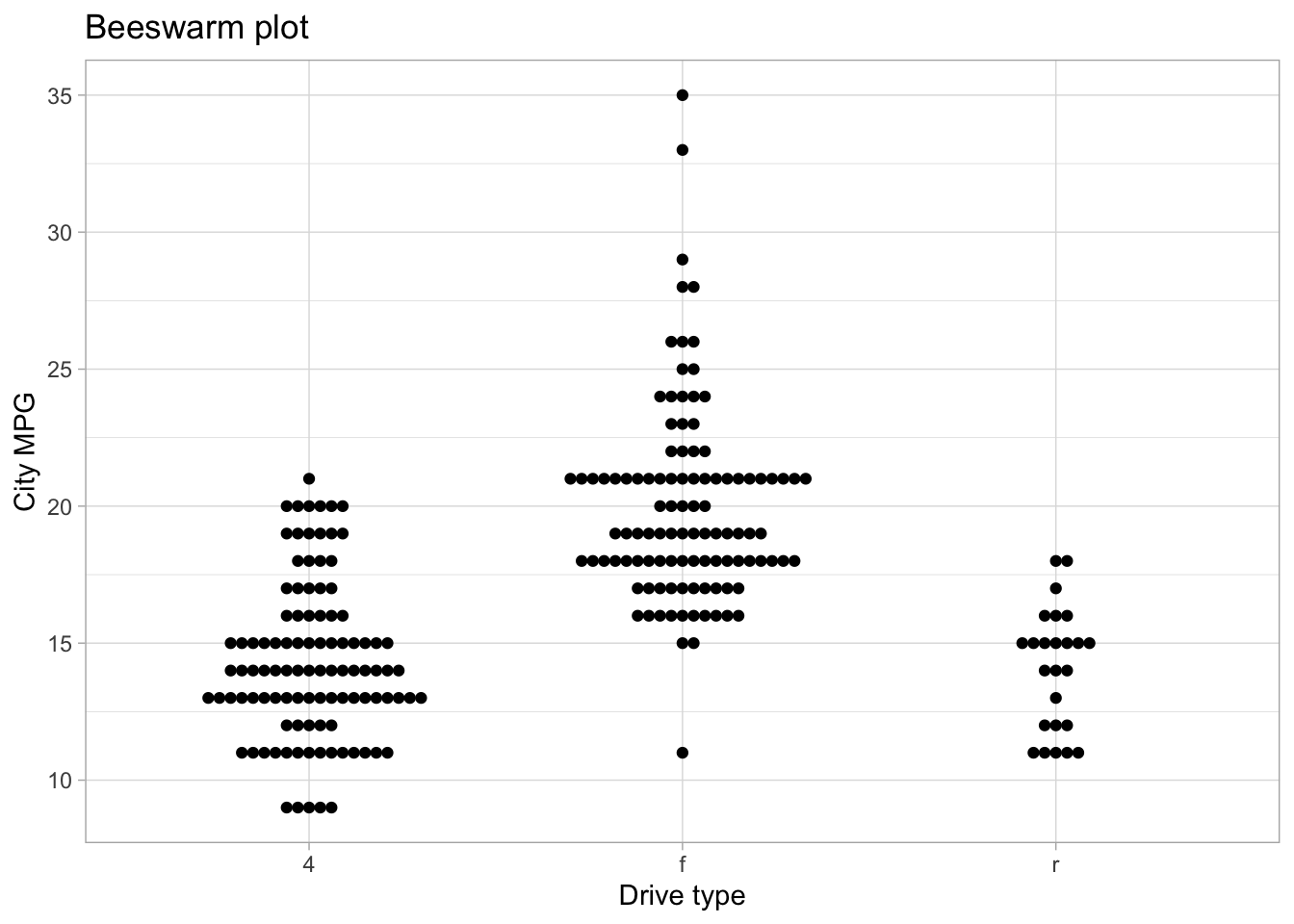### Categorical vs. categorical

For these kinds of plots, the most common choice is for something like a dodged bar plot or a stacked bar plot. Something like this:

ggplot(mpg, aes(x=drv, fill=ordered(cyl))) +
geom_bar(position="dodge") +
labs(x="Drive type", fill="Cylinders", title="Dodged bar chart")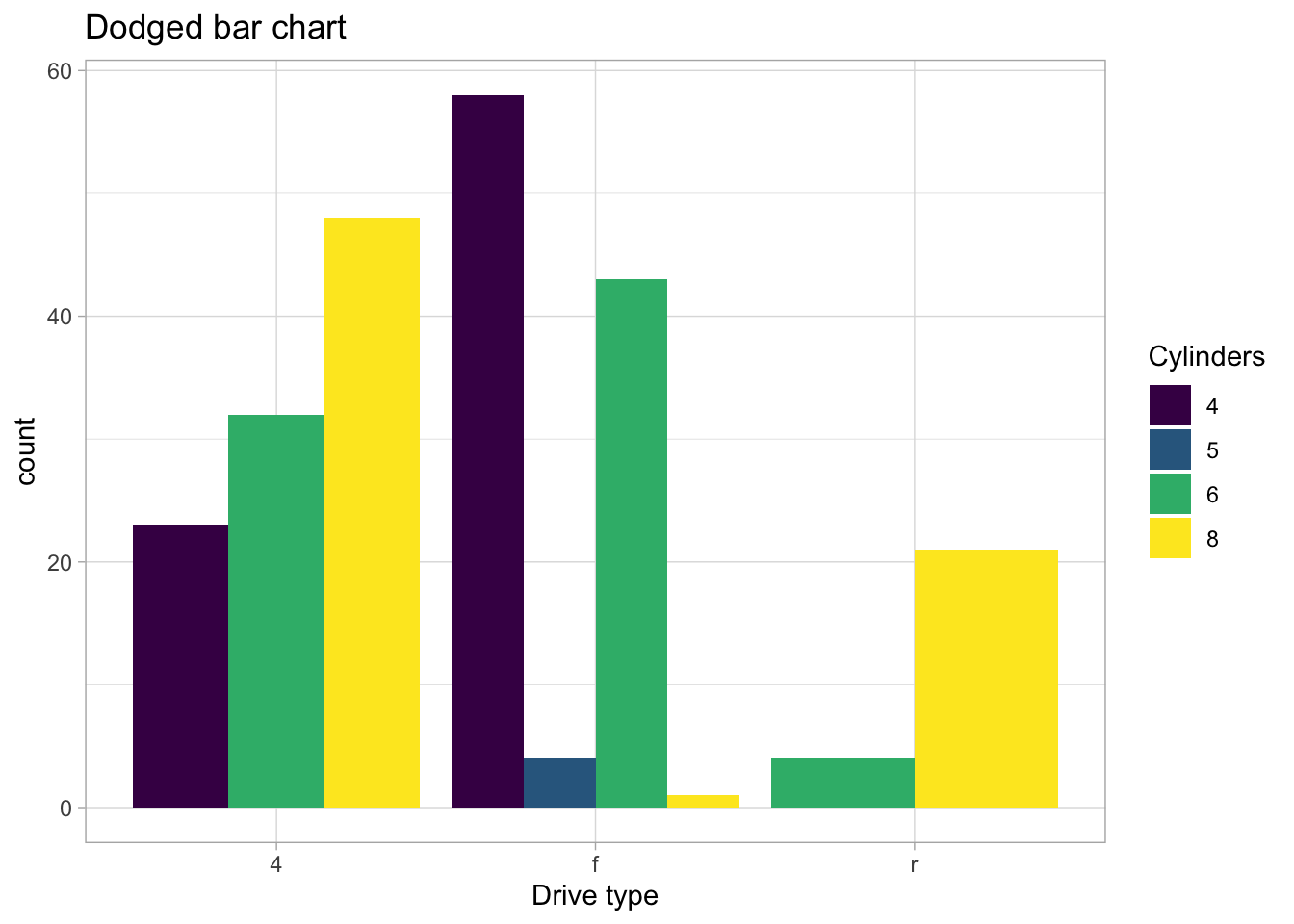ggplot(mpg, aes(x=drv, fill=ordered(cyl))) +
geom_bar() +
labs(x="Drive type", fill="Cylinders", title="Stacked bar chart")### Checking assumptions

The most common assumption we need to check with appropriate plots is the normality assumption - we do this with a probability plot or a quantile plot. In ggplot2, this is done with the geom_qq and geom_qq_line marks. These require, instead of connecting data to x or y, that data connects to sample.

Setting a color is automatically splitting the data into groups, so simultaneous probability plots can be done by setting a color. Alternatively, one could use facetting to get side-by-side probability-plots.

ggplot(mpg, aes(sample=cty)) +
geom_qq() + geom_qq_line() +
labs(title="Probability Plot for City MPG, all data")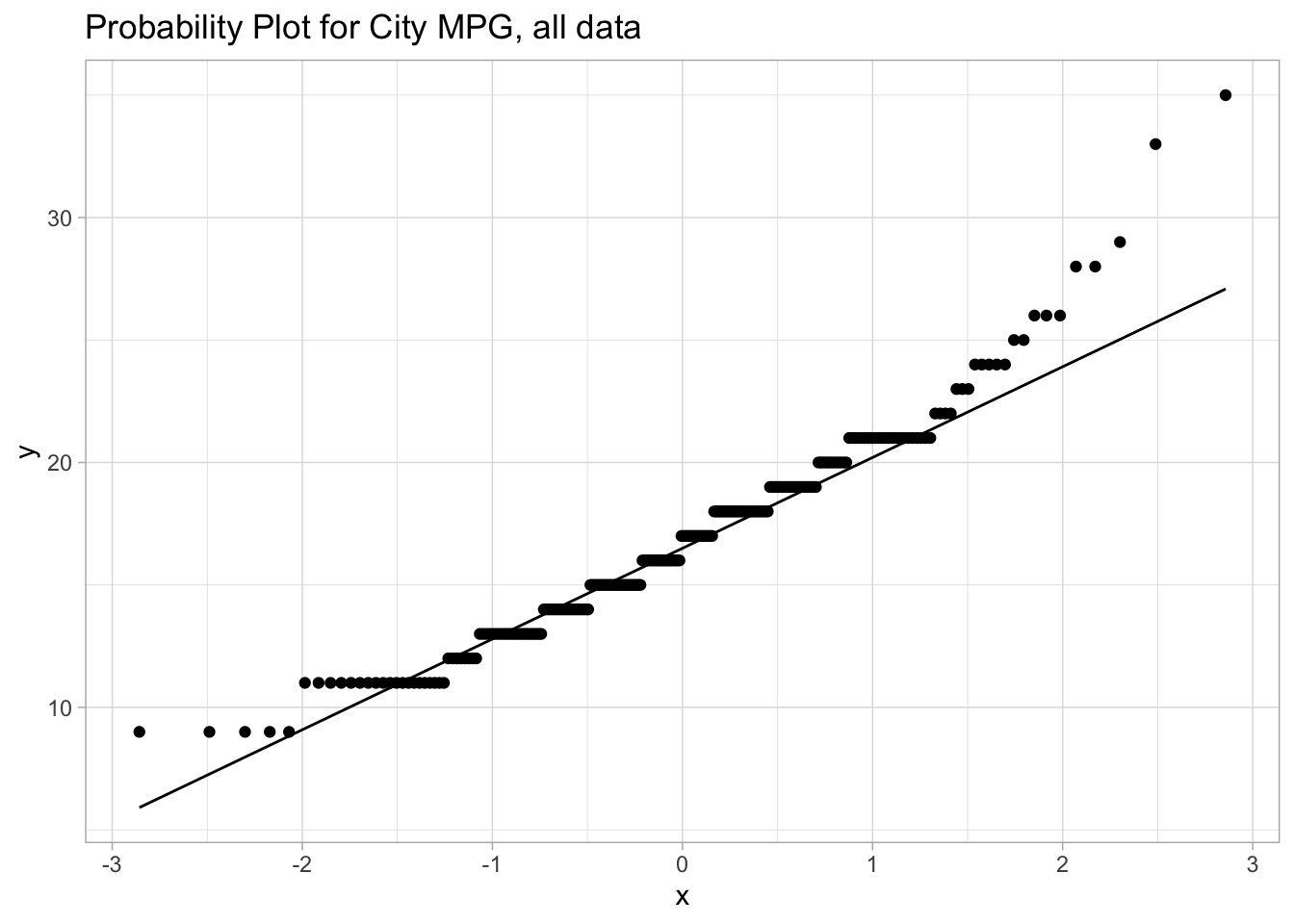ggplot(mpg, aes(sample=cty, color=drv)) +
geom_qq() + geom_qq_line() +
labs(title="Probability Plot for City MPG, split by Drive Type", color="Drive Type")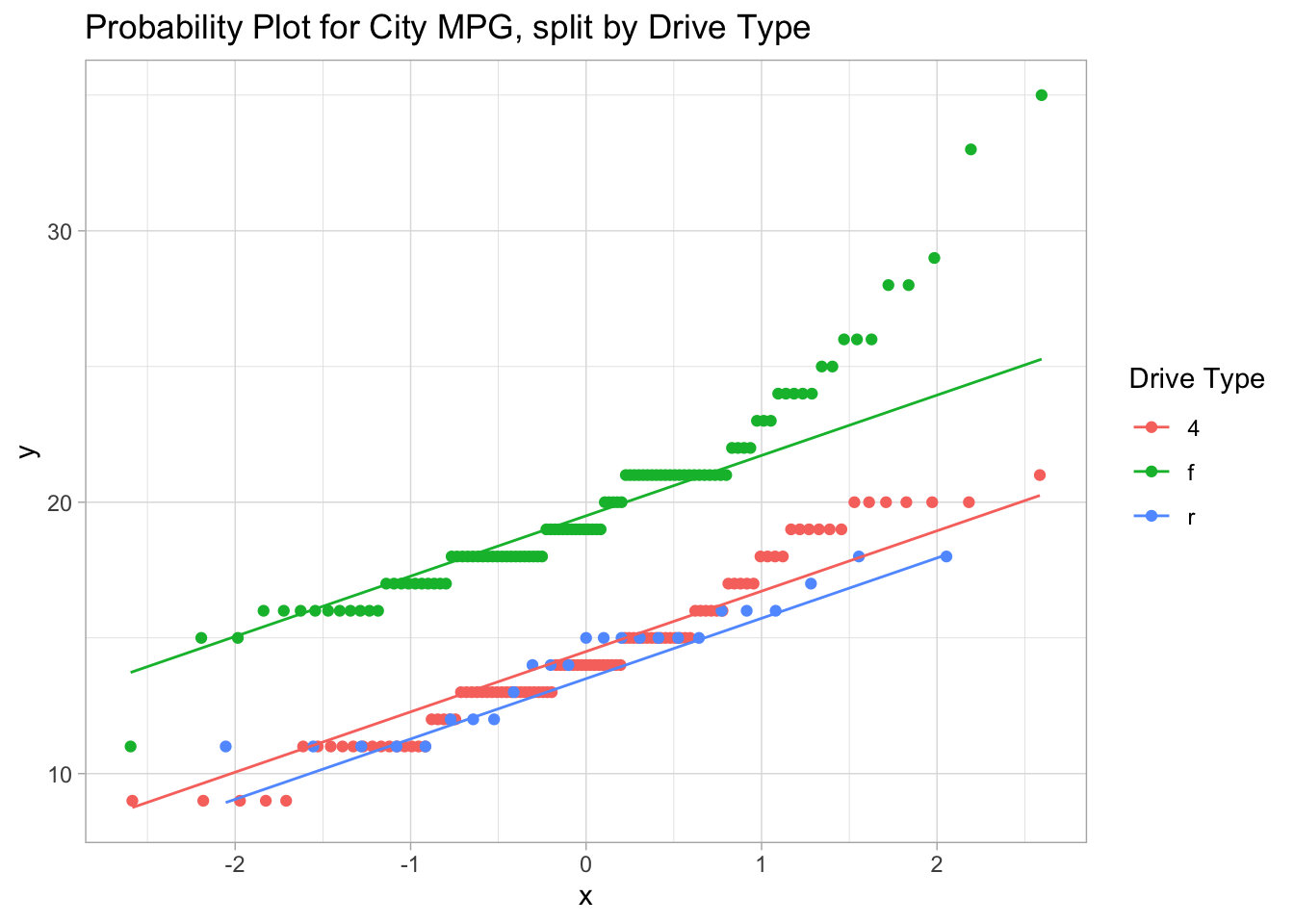ggplot(mpg, aes(sample=cty)) +
geom_qq() + geom_qq_line() +
facet_wrap(~drv) +
labs(title="Probability Plot for City MPG, split by Drive Type")